# Dipole fitting of combined MEG/EEG data

## Introduction

In this tutorial you can find information about how to fit dipole models to the event-related fields (MEG) and potentials (EEG) of a single subject. We will be working on the dataset described in the Preprocessing and event-related activity tutorial, and we will use the anatomical images that belong to the same subject. We will repeat some code here to select the trials, preprocess the data and compute averages that are suitable for dipole fitting. We assume that preprocessing and event-related averaging is already clear for the reader.

This tutorial will not show how to combine source-level data over multiple subjects. It will also not describe how to do source-localization of oscillatory activation. You can check the Localizing oscillatory sources using beamformer techniques tutorial if you are interested in the later.

This tutorial contains the hands-on material of the NatMEG workshop and is complemented by this lecture.

## Background

The EEG/MEG signals measured on or around the scalp do not directly reflect the activated neurons in the brain. To reconstruct the actual activity in the brain, source reconstruction techniques are used. You can read more about the different methods in the review papers that are listed here.

The activity in the brain is estimated from the EEG or MEG signals using

1. the EEG/MEG activity itself that is measured on or around the scalp
2. the spatial arrangement of the electrodes/gradiometers relative to the brain (sensor positions),
4. the location of the source (source model)

Using this information, source estimation comprises two major steps: (1) Estimation of the EEG potential or MEG field distribution for a known source is referred to as forward modeling. (2) Estimation of the unknown sources corresponding to the measured EEG or MEG is referred to as inverse modeling.

The forward solution can be computed when the head model, the sensor positions and a model for the source are given. For distributed source models and for scanning approaches such as beamforming, the source model consists of a discrete description of the the brain volume or of the cortical sheet in many voxels or vertices. When the forward solution is computed, the lead field matrix (with dimensions Nchan * Nsources) is calculated for each point, taking into account the head model and the sensor positions.

A prerequisite of forward modeling is that the geometrical description of the sensor positions, head model and source model are expressed in the same coordinate system (e.g., CTF, MNI, Talairach) and with the same units (mm, cm, or m). There are different conventions for coordinate systems. The precise coordinate system is not relevant, as long as all data is consistent. Here you can read how the different head and MRI coordinate systems are defined. For most MEG systems, the gradometer sensors are by default defined relative to head localizer coils or anatomical landmarks, therefore when the anatomical MRI are aligned to the same landmarks, the position of the MEG sensors directly matches the MRI. As EEG data is typically not explicitly aligned relatively to the head, therefore, the EEG electrodes usually have to be explicitly realigned prior to source reconstruction (see also this faq and this example).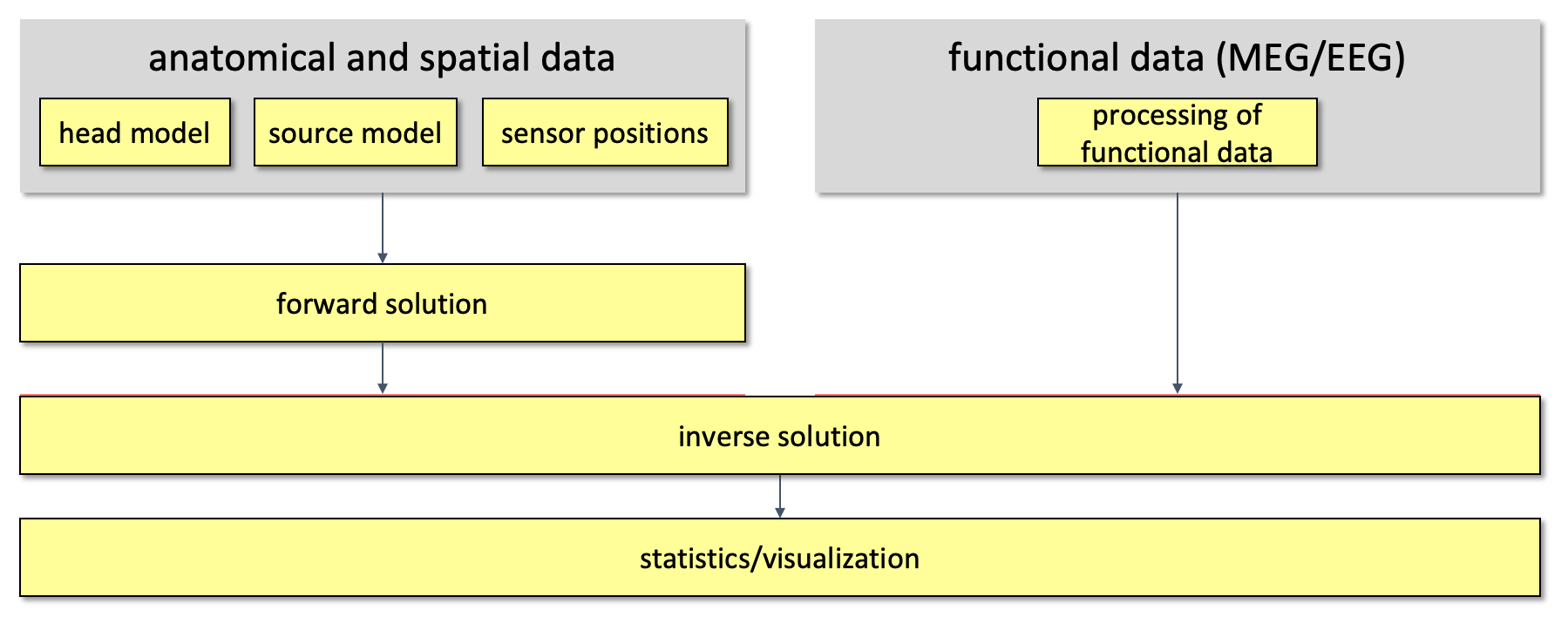Figure 1. Overall outline of the pipeline used for source reconstruction

In the Preprocessing and event-related activity tutorial, time-locked averages of event-related fields of the standard and deviant conditions were computed and it was shown that there is a difference between the conditions. The topographical distribution of the ERFs belonging to each condition to the difference have been plotted. The aim of this tutorial is to localise the sources of the underlying neuronal activity. For this we need a source model and a volume conduction model.

### Source model

In this tutorial we will use the dipole fitting approach (1) to localise the neuronal activity and (2) to estimate the time course of the activity. This approach is most suitable for relatively early cortical activity which is not spread over many or large cortical areas. Dipole fitting assumes that a small number of point-like equivalent current dipoles (ECDs) can describe the measured topography. It optimises the location, the orientation and the amplitude of the model dipoles in order to minimise the difference between the model and measured topography. A good introduction to dipole fitting is provided by Scherg (Source localization by fitting an equivalent current dipole model Scherg M. Fundamentals of dipole source potential analysis. In: Auditory evoked magnetic fields and electric potentials. eds. F. Grandori, M. Hoke and G.L. Romani. Advances in Audiology, vol. 6. Karger, Basel, pp 40-69, 1990).

### Volume conduction model

A volume conduction model of the head, also known as a head model, is represented in FieldTrip as a MATLAB structure. It describes how the currents flow through the tissue, not where they originate from. In general it consists of a description of the geometry of the tissue(s), a description of the conductivity of the tissue(s), and mathematical parameters that are derived from these. How the mathematical parameters are described depends on the computational solution to the forward problem, either by numerical approximations (e.g., using the boundary element and finite element method, or BEM and FEM), or by exact analytical solutions (e.g., for spherical models).

The more accurate the description of the geometry of the head or the source, the better the quality of the forward model. There are many types of head models which, to various degrees, take the individual anatomy into account. The different head models available in FieldTrip are listed here.

## Procedure

To fit the dipole models to the data, we will perform the following steps:

• We will preprocess the anatomical images in MATLAB. First, the mri image is read in with ft_read_mri, then the mri is aligned with the MEG data using ft_volumerealign, and subsequently it is resliced with ft_volumereslice to ensure that the volume is isotropic and to align the volume with the cardinal axes of the coordinate system.
• The resliced volume is segmented to obtain the anatomical description of the brain, skull and skin with ft_volumesegment.
• After creating meshes with the triangulated description of the outer brain, skull and skin compartment with ft_prepare_mesh, we create a volume conduction model using ft_prepare_headmodel;
• We preprocess the MEG and EEG data using ft_definetrial and ft_preprocessing and compute the average over trials using ft_timelockanalysis.
• Using ft_dipolefitting we will fit dipole models to the averaged data for each condition and to the difference between the conditions.
• Throughout this tutorial, we will use the high-level plotting functions to look at the data, and some lower-level plotting functions to make detailled visualizations.

### Read and visualise the anatomical data

We start with the anatomical MRI data, which comes directly from the scanner in DICOM format. You can download the dicom.zip from our download server. We suggest that you unzip the dicom files in a separate directory.

DICOM datasets consist of a large number of files, one per slice. As filename you have to specify a single file, the reading function will automatically determine which other slices are part of the same anatomical volume and put them in the correct order.

``````mrifile = './dicom/00000113.dcm';
``````

``````dataset = 'oddball1_mc_downsampled.fif';

``````

The high-level plotting functions do not offer support for flexible plotting of the geometrical information. The plotting module, i.e. the set of functions in the fieldtrip/plotting directory, includes a number of lower-level functions to make nice figures of the various geometrical data objects. In contrast to the high-level functions, these plotting functions do not take a cfg as first input argument.

``````figure
ft_plot_sens(elec, 'style', '*g');

view([1 0 0])
print -dpng natmeg_dip_geometry1.png
``````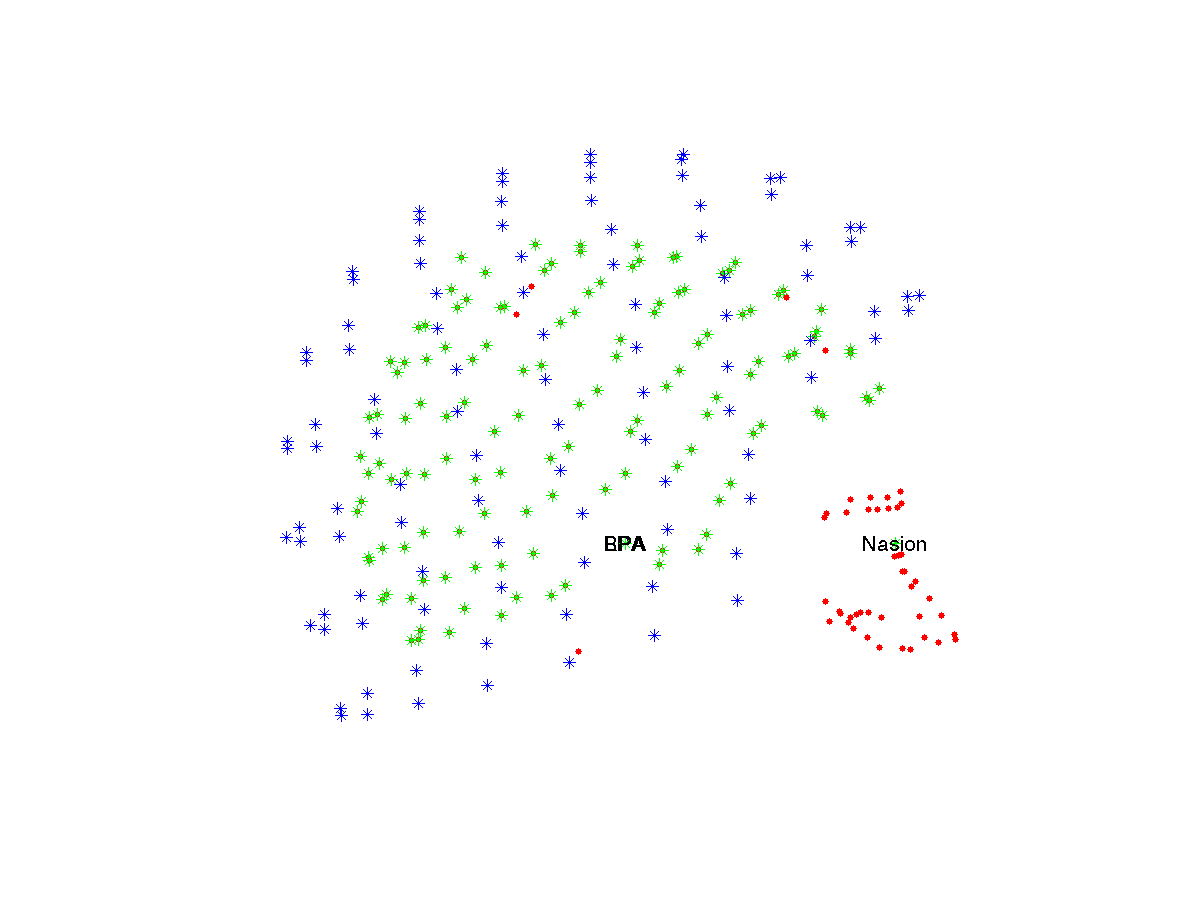It is possible to visualise the anatomical MRI using the ft_sourceplot function. Usually we use the function to overlay functional data from a beamformer source reconstruction on the anatomical MRI, but in the absence of the functional data it will simply show the anatomical MRI. Besides showing the MRI, you can also use the function to see how the MRI is aligned with the coordinate system, and how the voxel indices [i j k] map onto geometrical coordinates [x y z].

``````figure
cfg = [];
ft_sourceplot(cfg, mri_orig);

save mri_orig mri_orig
``````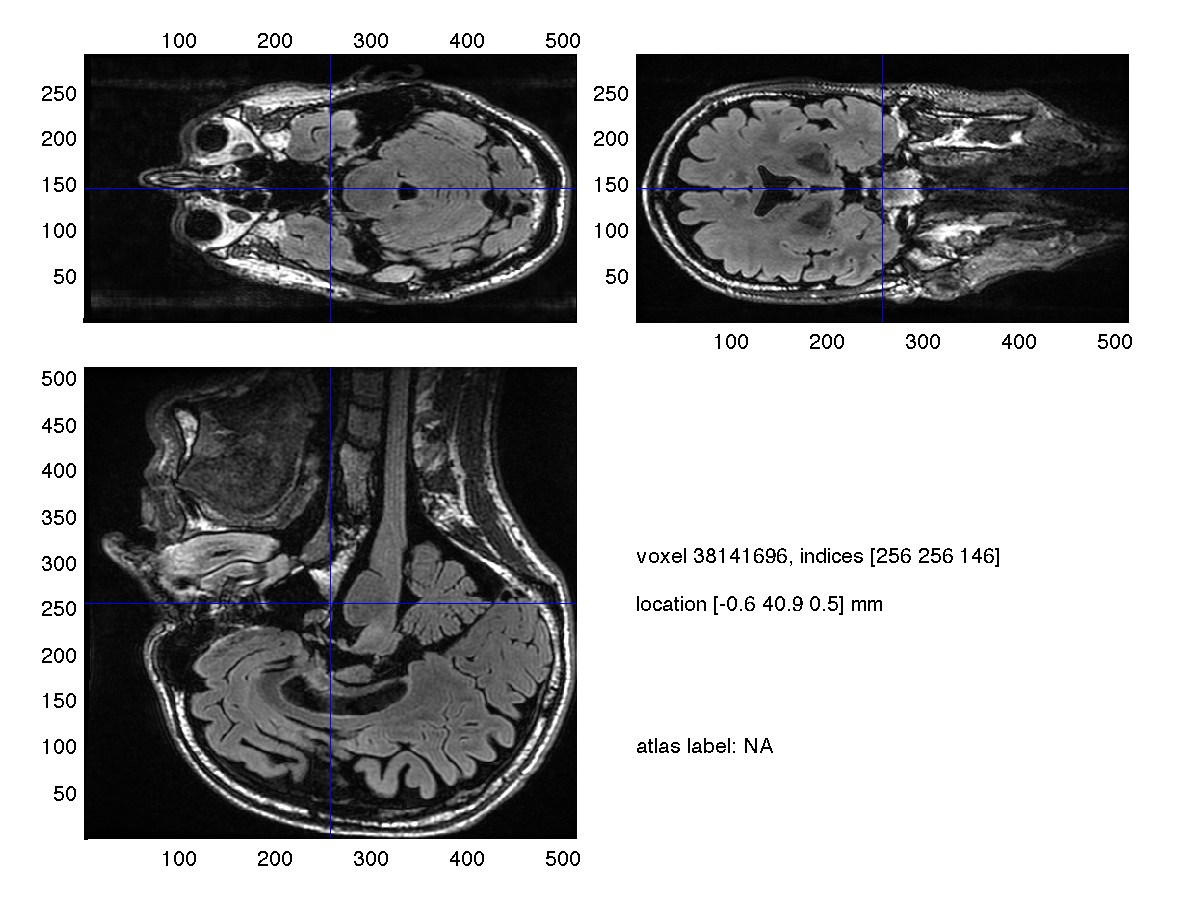You can see that the MRI is displayed upside down. That in itself is not a problem, as long as the coordinate system correctly describes the MRI. This frequently asked question explains why it is not a problem. However, if you click around in the MRI and look how the [x y z] position in the lower right panel is updated, you should recognize that the MRI is not coregistered with the Neuromag head coordinate system.

### Coregister the anatomical MRI to the MEG coordinate system

The coregistration of the anatomical MRI with the Neuromag head coordinate system is required to express the anatomical MRI in a consistent fashion relative to the MEG and EEG sensors. Since we will use the anatomical MRI to construct the volume conduction model of the head, coregistration is also a prerequisite to ensure that the volume conduction model is aligned with the sensors.

The first step consists of a coarse coregistration, based on three anatomical landmarks at the nasion (i.e. at the top of the bridge of the nose) and two pre-auricular points. We use ft_volumerealign with cfg.method=’interactive’. It allows us to click on a voxel, and to press ‘n’, ‘l’ or ‘r’ to indicate the nasion, left and right pre-auricular point respectively.

``````cfg = [];
cfg.method = 'interactive';
cfg.coordsys = 'neuromag';
[mri_realigned1] = ft_volumerealign(cfg, mri_orig);

save mri_realigned1 mri_realigned1
``````

It is difficult to precisely determine the position of the pre auricular points. One solution therefore is to use markers that are visible in the MRI, which is the strategy we commonly emply at the Donders Institute. The alternative, which is often used at 4D/BTi and Neuromag sites, is to record the shape of the head using a Polhemus electromagnetic tracker. The Polhemus head shape and the skin surface that is extracted from the MRI are subsequently coregistered.

``````cfg = [];
[mri_realigned2] = ft_volumerealign(cfg, mri_realigned1);
``````

The headshape based coregistration starts with an interactive step to improve the alignment of the MRI-derived head shape with the Polhemus points. You should specify the translation and rotation in the graphical user interface. Subsequently an automatic iterative-closest-points algorithm is used to fine-tune the coregistration.

``````save mri_realigned2 mri_realigned2
``````

Check once more with ft_sourceplot whether the coordinate system is consistent with the MRI. Is the problem of the MRI being upside down resolved? Is the coordinate system correct?

We reslice the MRI on to a 1x1x1 mm cubic grid which is aligned with the coordinate axes. This is not only convenient for plotting, but we also need it later on for the imerode/imdilate image processing functions.

``````cfg = [];
cfg.resolution = 1;
cfg.xrange = [-100 100];
cfg.yrange = [-110 140];
cfg.zrange = [-80 120];
mri_resliced = ft_volumereslice(cfg, mri_realigned2);

save mri_resliced mri_resliced

figure
ft_sourceplot([], mri_resliced);
print -dpng natmeg_dip_mri_resliced.png
``````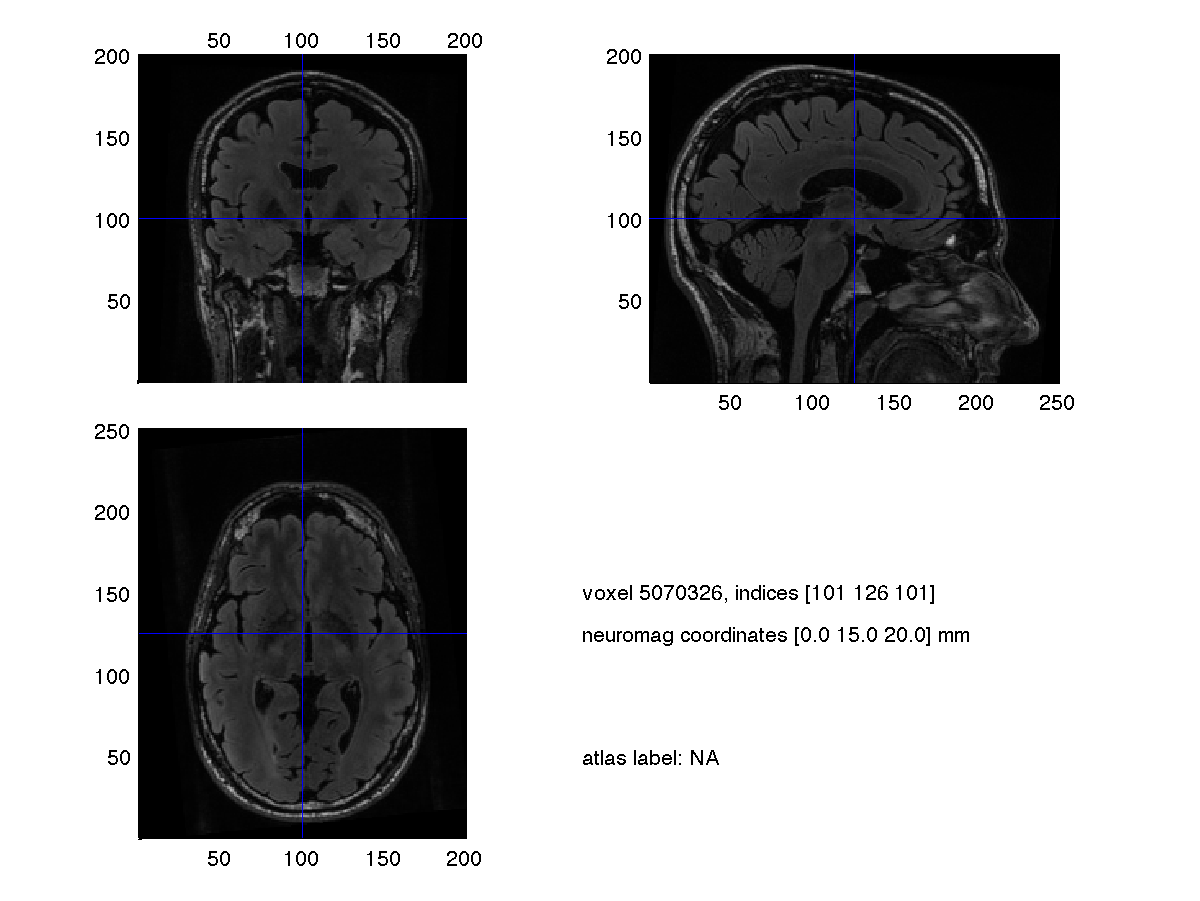``````% the low-level plotting functions do not know how to deal with units,
% so make sure we have the MRI expressed in cm as well
mri_resliced_cm = ft_convert_units(mri_resliced, 'cm');

save mri_resliced_cm mri_resliced_cm
``````

### Construct the MEG volume conduction model

Now that we have the anatomical MRI coregistered and resliced in to isotropic voxels, we proceed and segment the brain, skull and scalp tissue.

``````cfg           = [];
cfg.output    = {'brain', 'skull', 'scalp'};
mri_segmented = ft_volumesegment(cfg, mri_resliced);

% copy the anatomy into the segmented mri
mri_segmented.anatomy = mri_resliced.anatomy;

save mri_segmented mri_segmented
``````

By treating the segmentation of brain/skull/scalp as a “functional” volume, we can trick ft_sourceplot into plotting it on top of the anatomical MRI.

``````cfg = [];
cfg.funparameter = 'brain';
ft_sourceplot(cfg, mri_segmented);
print -dpng natmeg_dip_segmented_brain.png

cfg.funparameter = 'skull';
ft_sourceplot(cfg, mri_segmented);
print -dpng natmeg_dip_segmented_skull.png

cfg.funparameter = 'scalp';
ft_sourceplot(cfg, mri_segmented);
print -dpng natmeg_dip_segmented_scalp.png
``````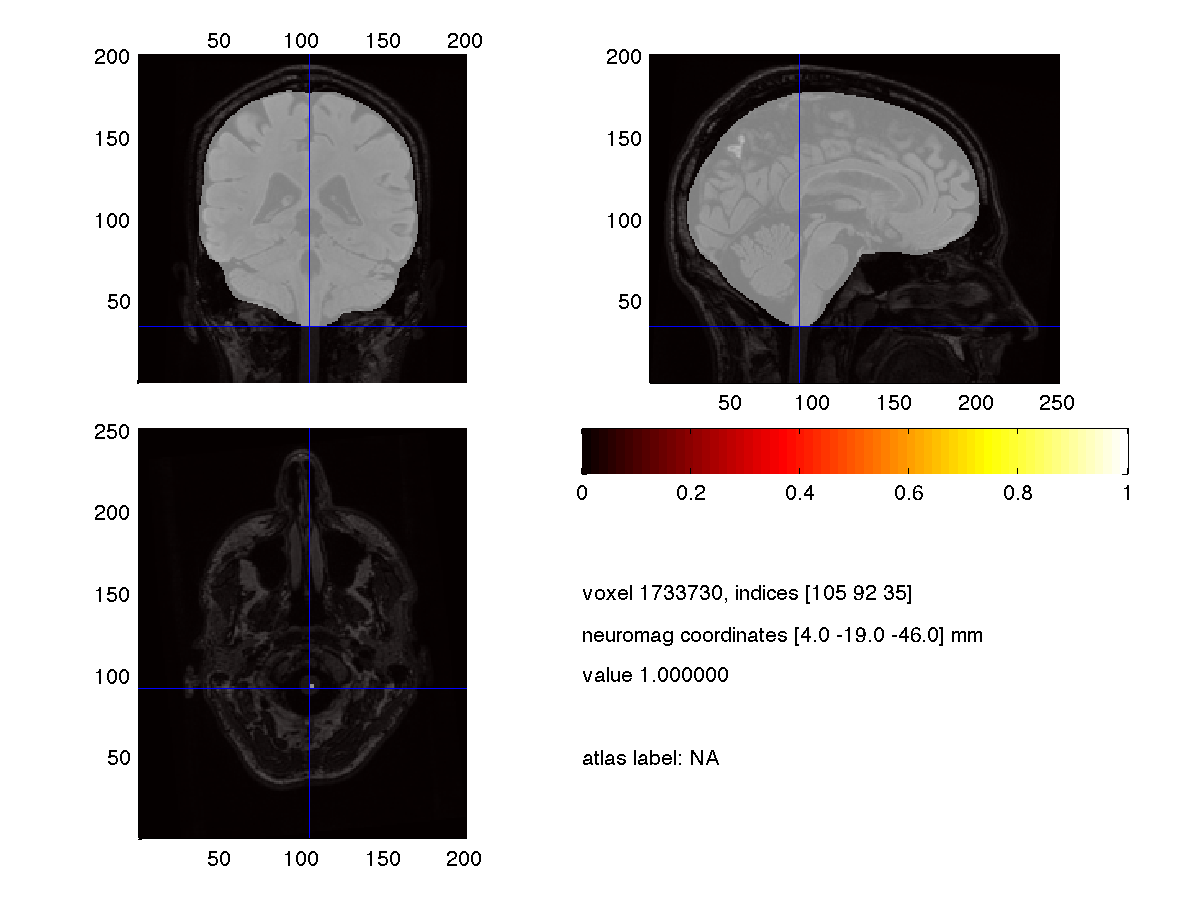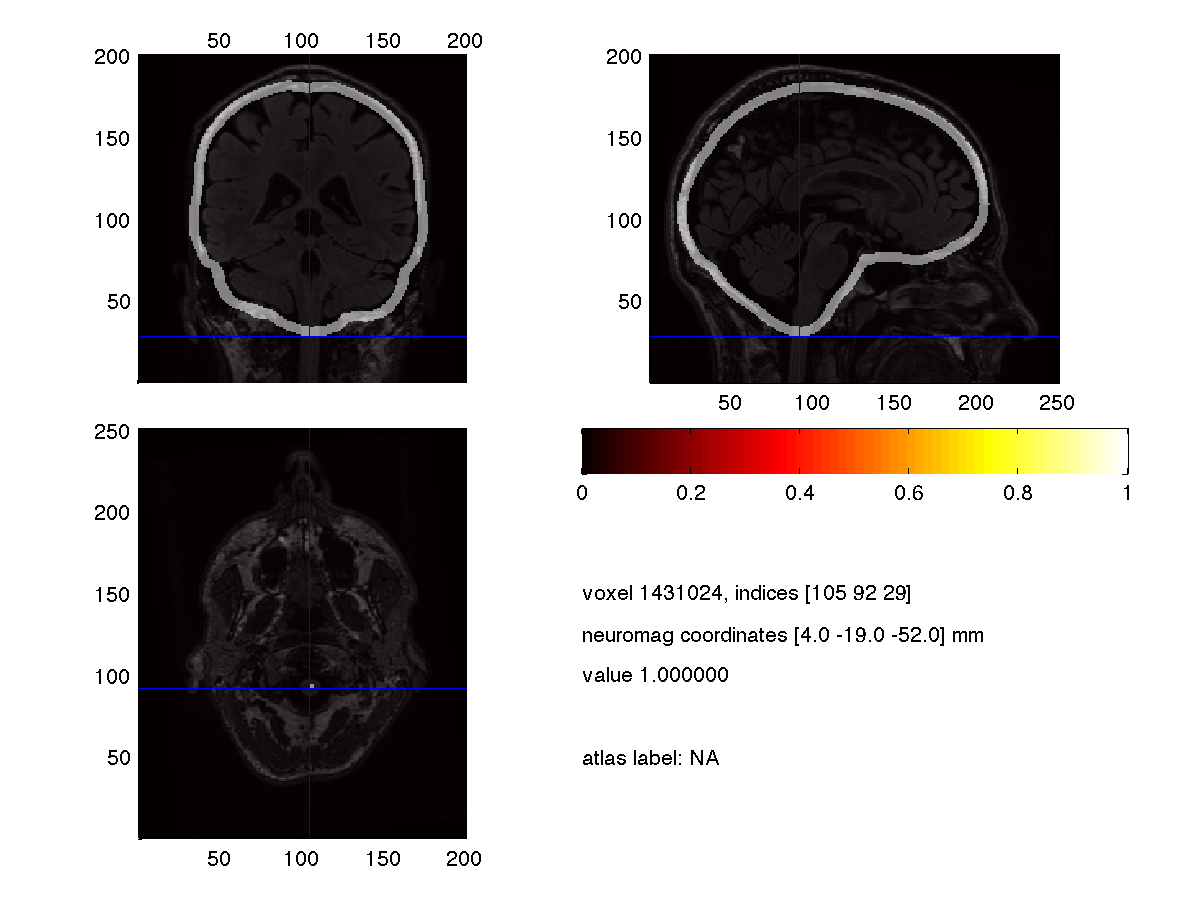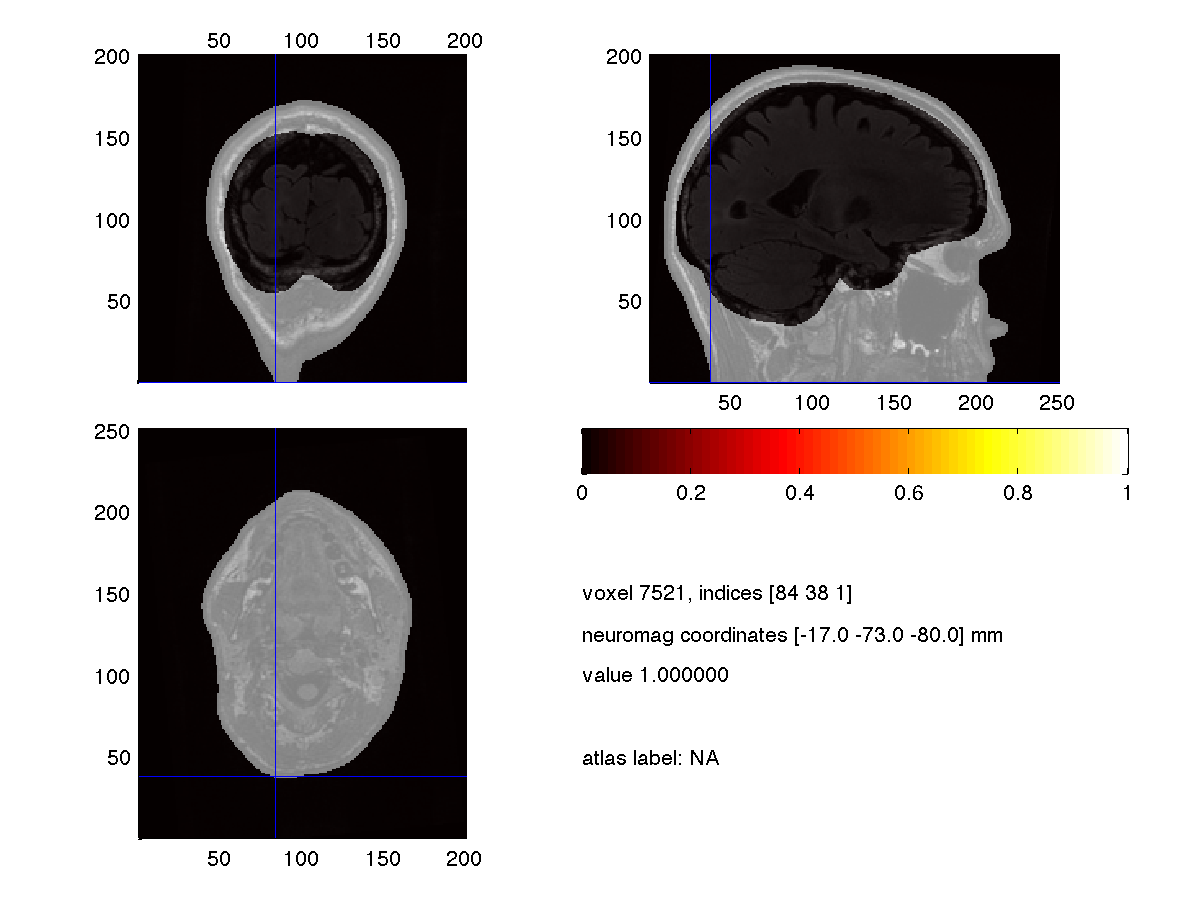You should check that the segmentation covers the appropriate part of the anatomical MRI and that it does not have any artefacts due to noisy voxels in the MRI or local contrast drop-out.

After having confirmed that the segmentations are consistent with the anatomical MRI, we construct triangulated meshes to describe the outside of each segmented volume.

``````cfg = [];
cfg.method = 'projectmesh';
cfg.tissue = 'brain';
cfg.numvertices = 3000;
mesh_brain = ft_prepare_mesh(cfg, mri_segmented);

cfg = [];
cfg.method = 'projectmesh';
cfg.tissue = 'skull';
cfg.numvertices = 2000;
mesh_skull = ft_prepare_mesh(cfg, mri_segmented);

cfg = [];
cfg.method = 'projectmesh';
cfg.tissue = 'scalp';
cfg.numvertices = 1000;
mesh_scalp = ft_prepare_mesh(cfg, mri_segmented);
``````

Why do we use fewer vertices for the outer mesh than for the inner mesh?

These meshes are all relatively coarse and don’t look so nice in a visualization. Using the isosurface method (also known as Marching Cubes) we can extract a much nicer looking skin conpartment.

``````cfg = [];
cfg.method = 'isosurface';
cfg.tissue = 'scalp';
cfg.numvertices = 16000;
highres_scalp = ft_prepare_mesh(cfg, mri_segmented);

save mesh mesh_* highres_scalp

figure
ft_plot_mesh(mesh_scalp, 'edgecolor', 'none', 'facecolor', 'skin')
material dull
camlight
lighting phong
print -dpng natmeg_dip_scalp.png
``````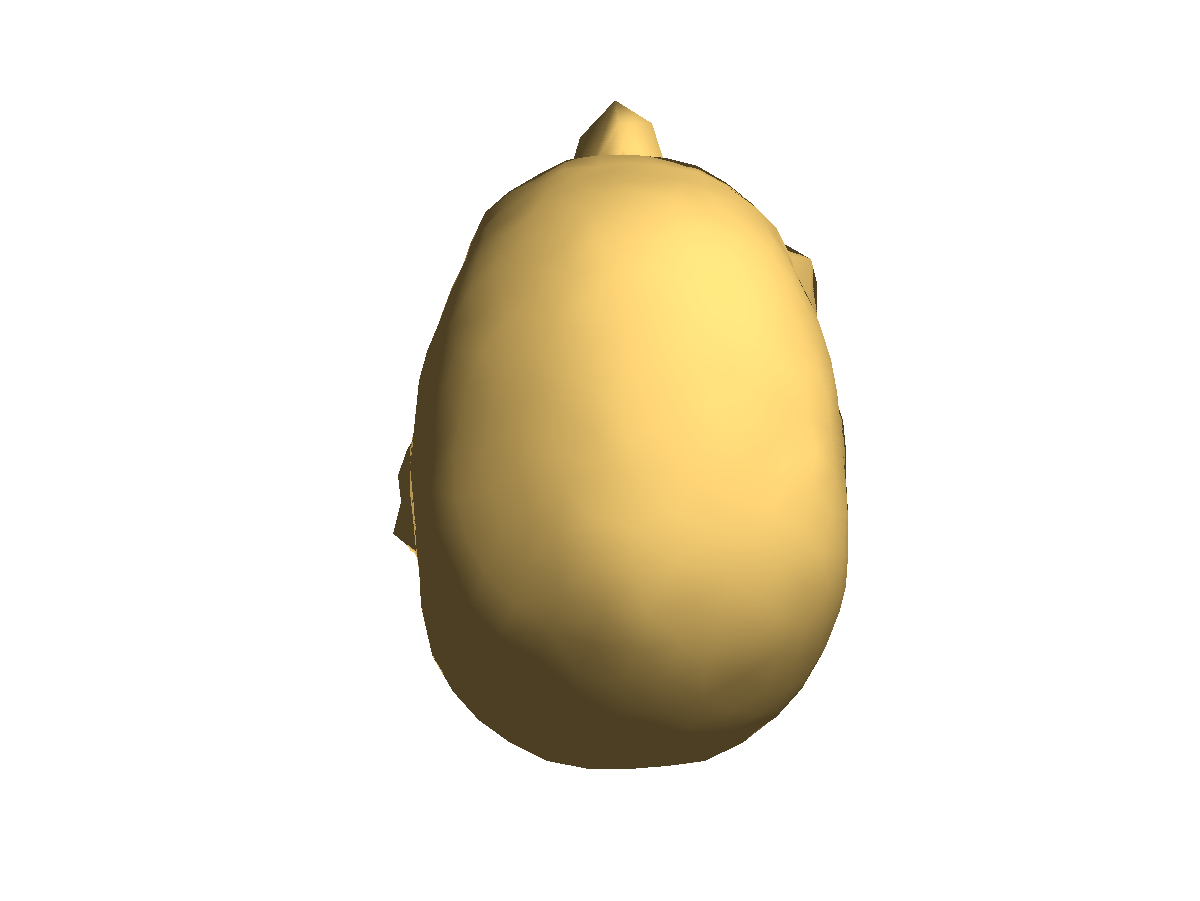``````figure
ft_plot_mesh(highres_scalp, 'edgecolor', 'none', 'facecolor', 'skin')
material dull
camlight
lighting phong
print -dpng natmeg_dip_highres_scalp.png
``````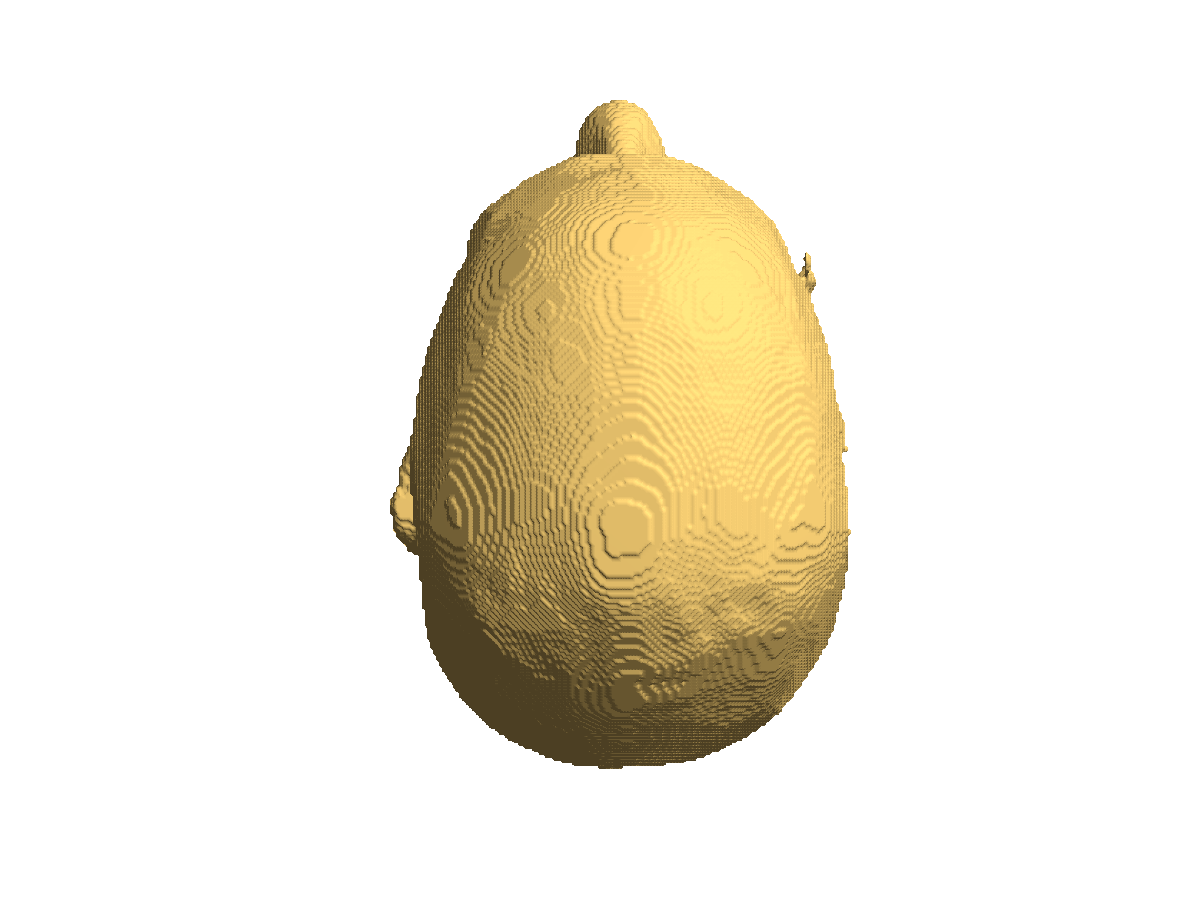You can type “camlight” multiple times, to get light from various directions.

It is also convenient to switch on the “Camera Toolbar” (under the figure menu -> View).

Using the rotate3d command, or the corresponding button in the toolbar, you can rotate the mesh in the figure with your mouse.

Now that we have the meshes, we use them to compute the volume conduction model. For the MEG, only the mesh that describes the interface between the brain and the skull is relevant.

``````cfg = [];
cfg.method = 'singleshell';

figure
hold on
ft_plot_sens(elec, 'style', 'og');
ft_plot_headmodel(headmodel_meg, 'facealpha', 0.5, 'edgecolor', 'none'); % "lighting phong" does not work with opacity
material dull
camlight

view([1 0 0])
print -dpng natmeg_dip_geometry2.png
``````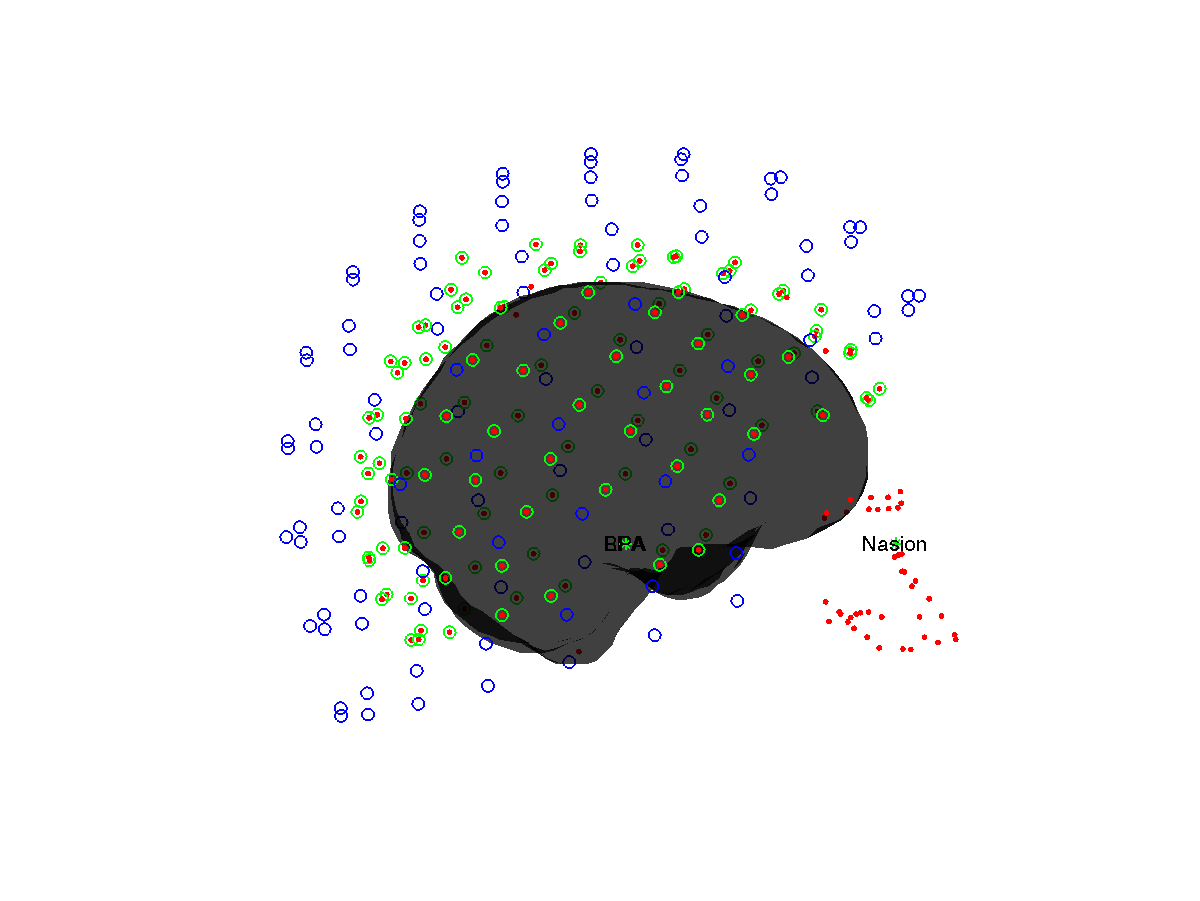### Process the MEG data

The processing of the MEG dataset is done similar to the Preprocessing and event-related activity in MEG and EEG data tutorial. It requires the custom trial function trialfun_oddball_stimlocked.m to be on your MATLAB path.

#### Segment and read the MEG data

``````cfg = [];
cfg.dataset = dataset;
cfg.trialdef.prestim        = 0.2;
cfg.trialdef.poststim       = 0.4;
cfg.trialdef.rsp_triggers   = [256 4096];
cfg.trialdef.stim_triggers  = [1 2];
cfg.trialfun                = 'trialfun_oddball_stimlocked';

cfg = ft_definetrial(cfg);

cfg.continuous    = 'yes';
cfg.hpfilter      = 'no';
cfg.detrend       = 'no';
cfg.demean        = 'yes';
cfg.baselinewindow = [-inf 0];
cfg.dftfilter     = 'yes';
cfg.dftfreq       = [50 100];
cfg.lpfilter      = 'yes';
cfg.lpfreq        = 120;
cfg.channel       = 'MEG';
cfg.precision     = 'single';

data_meg = ft_preprocessing(cfg);

save data_meg data_meg
``````

We screen for bad trials using ft_rejectvisual. Using your mouse, you can click-and-drag in the lower left figure to select trials that are to be removed.

``````cfg = [];
cfg.method = 'summary';
cfg.channel = 'MEG*1';
cfg.keepchannel = 'yes';
data_meg_clean1 = ft_rejectvisual(cfg, data_meg);

cfg.channel = {'MEG*2', 'MEG*3'};
data_meg_clean2 = ft_rejectvisual(cfg, data_meg_clean1);

save data_meg_clean2 data_meg_clean2
``````

#### Compute the time-locked average

Using the trialinfo field, which contains the trigger code, the response code and the reaction time, we can select the standard and the deviant trials and compute a time-locked ER

``````cfg = [];
timelock_all = ft_timelockanalysis(cfg, data_meg_clean2);

cfg.trials = find(data_meg_clean2.trialinfo==1);
timelock_std = ft_timelockanalysis(cfg, data_meg_clean2);

cfg.trials = find(data_meg_clean2.trialinfo==2);
timelock_dev = ft_timelockanalysis(cfg, data_meg_clean2);

cfg = [];
cfg.layout = 'neuromag306all.lay';
cfg.layout = 'neuromag306planar.lay';
cfg.layout = 'neuromag306mag.lay';
% cfg.channel = 'MEG*1';
% cfg.channel = {'MEG*2', 'MEG*3'};
ft_multiplotER(cfg, timelock_std, timelock_dev);

print -dpng natmeg_dip_meg_multiplot.png
``````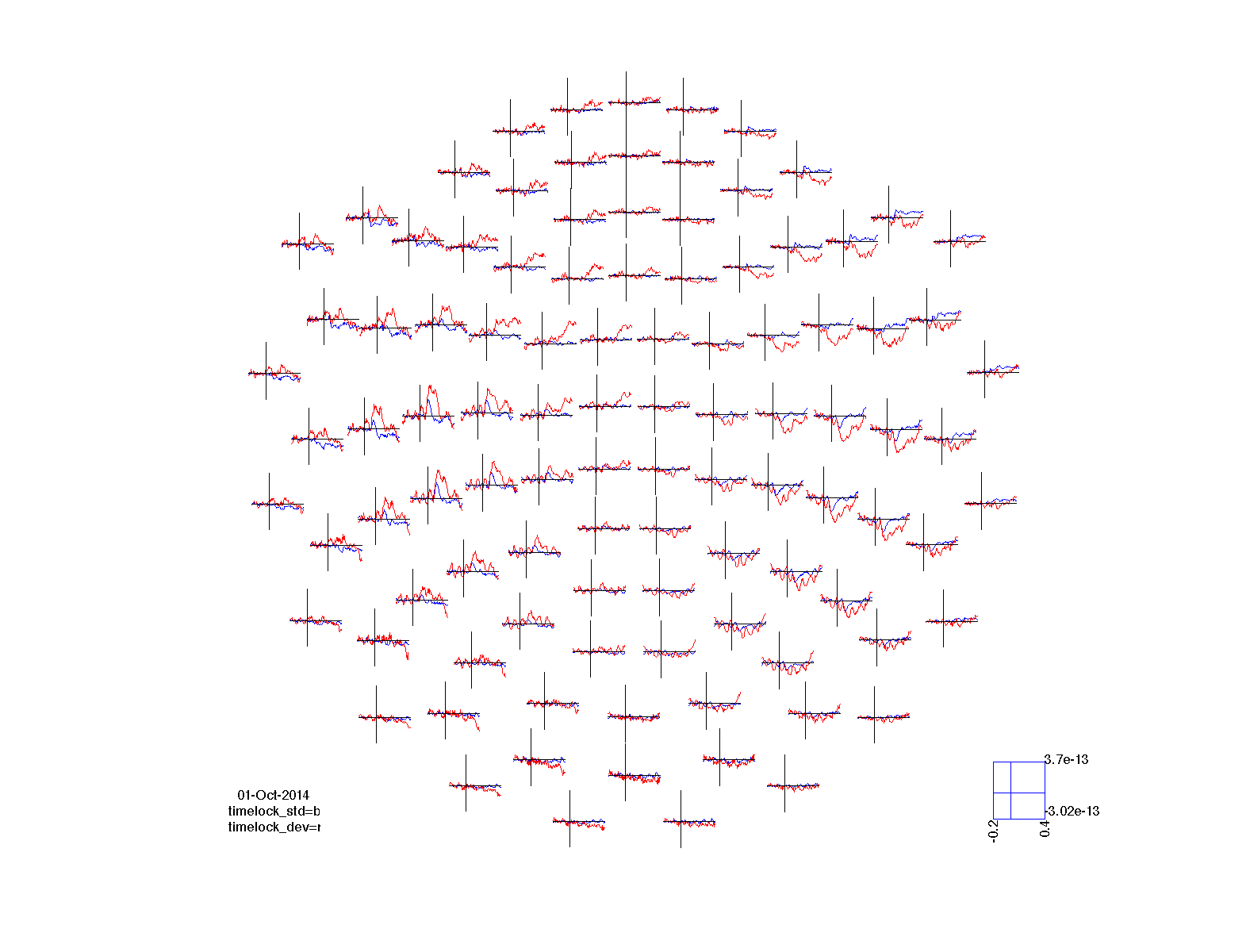As before, we also compute the difference waveform, i.e. the mismatch negativity.

``````cfg = [];
cfg.parameter = 'avg';
cfg.operation = 'x1 - x2';
timelock_dif = ft_math(cfg, timelock_dev, timelock_std);

cfg = [];
cfg.layout = 'neuromag306all.lay';
cfg.layout = 'neuromag306planar.lay';
cfg.layout = 'neuromag306mag.lay';
% cfg.channel = 'MEG*1';
% cfg.channel = {'MEG*2', 'MEG*3'};
ft_multiplotER(cfg, timelock_dif);

save timelock timelock*
``````

### Fit a dipole model to the MEG data

Having constructed the volume conduction model and completed the processing of the channel level data, we can investigate how well the data can be modeled with an Equivalent current Dipole (ECD) model. Since we expect activity in both auditory cortices, we will use a two-dipole model. Scanning the whole brain with two separate dipoles is not possible, but we can also start with the assumtion that the two dipoles are symmetric. In the Neuromag coordinate system the x-axis runs from the right to the left, hence we specify symmetry along the x-direction.

``````cfg = [];
cfg.latency = [0.080 0.110];
cfg.numdipoles = 2;
cfg.symmetry = 'x';
cfg.resolution = 1;
cfg.unit = 'cm';
cfg.gridsearch = 'yes';
cfg.senstype = 'meg';
cfg.channel = {'MEG*2', 'MEG*3'};
source_planar = ft_dipolefitting(cfg, timelock_all);

cfg.channel = 'MEG*1';
source_mag = ft_dipolefitting(cfg, timelock_all);
``````

Inspect the content of the source_mag structure. Can you identify where the position of the two dipoles is represented? And the orientation?

We can use ft_sourceplot to plot the cross-section of the MRI at the location of the first dipole.

``````cfg = [];
cfg.location = source_planar.dip.pos(1,:);
ft_sourceplot(cfg, mri_resliced_cm);

print -dpng natmeg_dip_planarortho.png
``````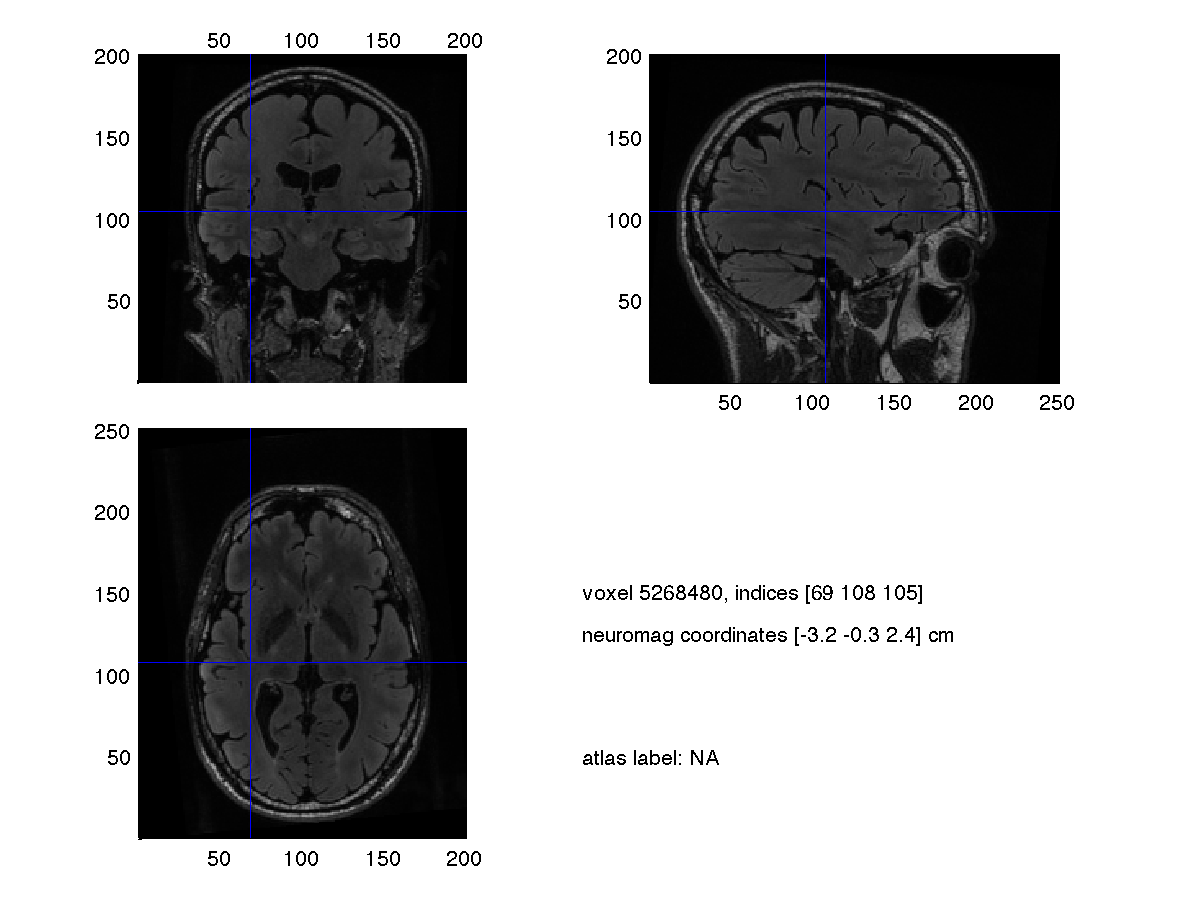This does not offer much insight in the two dipoles. Hence we again resort to the low-level plotting functions to make a 3-D figure that includes both dipoles and some select slices of the anatomical MRI.

``````figure
hold on

ft_plot_dipole(source_mag.dip.pos(1,:), mean(source_mag.dip.mom(1:3,:),2), 'color', 'r')
ft_plot_dipole(source_mag.dip.pos(2,:), mean(source_mag.dip.mom(4:6,:),2), 'color', 'r')

ft_plot_dipole(source_planar.dip.pos(1,:), mean(source_planar.dip.mom(1:3,:),2), 'color', 'g')
ft_plot_dipole(source_planar.dip.pos(2,:), mean(source_planar.dip.mom(4:6,:),2), 'color', 'g')

pos = mean(source_mag.dip.pos,1);
ft_plot_slice(mri_resliced_cm.anatomy, 'transform', mri_resliced_cm.transform, 'location', pos, 'orientation', [1 0 0], 'resolution', 0.1)
ft_plot_slice(mri_resliced_cm.anatomy, 'transform', mri_resliced_cm.transform, 'location', pos, 'orientation', [0 1 0], 'resolution', 0.1)
ft_plot_slice(mri_resliced_cm.anatomy, 'transform', mri_resliced_cm.transform, 'location', pos, 'orientation', [0 0 1], 'resolution', 0.1)

ft_plot_crosshair(pos, 'color', [1 1 1]/2);

axis tight
axis off

view(12, -10)
print -dpng natmeg_dip_symx.png
``````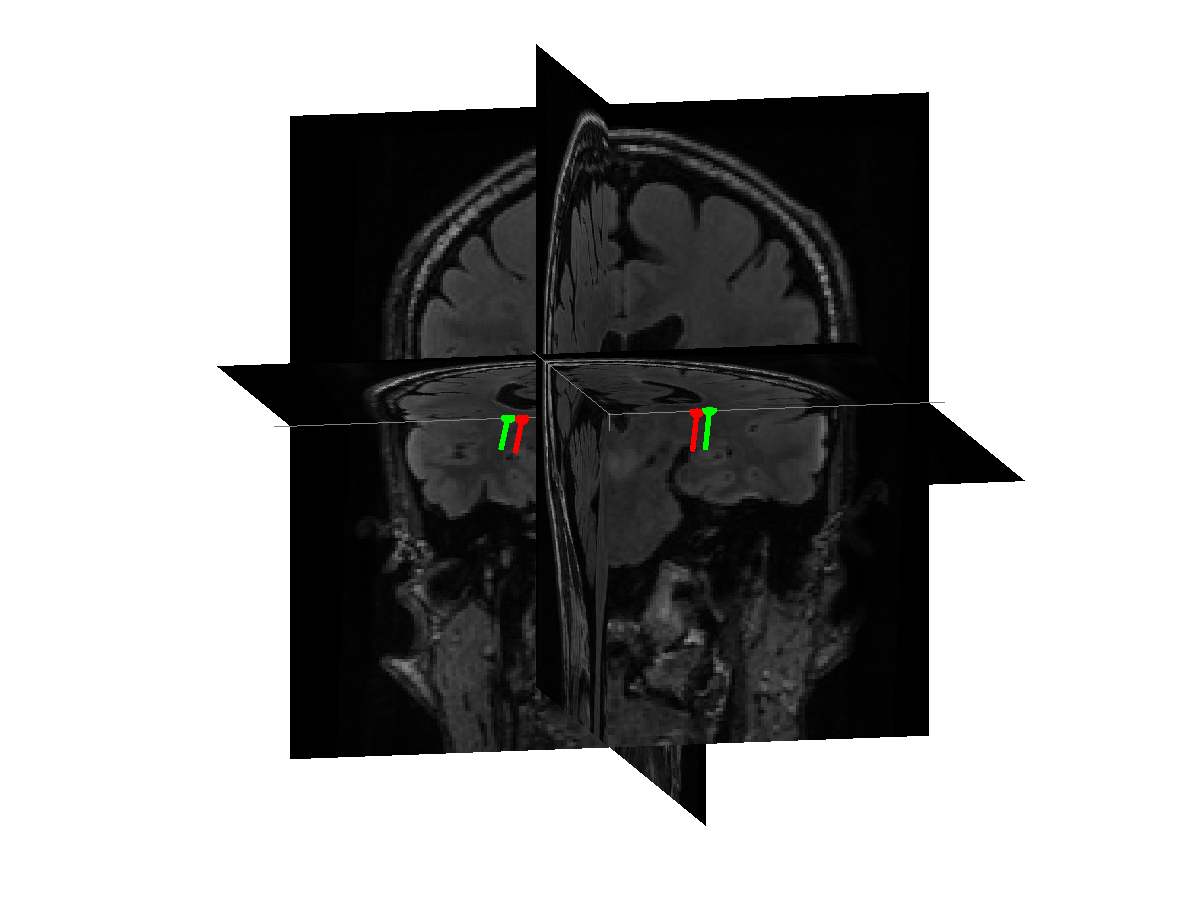Use the rotate functionality to get a 3-D impression of the location of the dipoles relative to the brain. The cross-section in the MRI is made at the average position of the two (symmetric) dipoles and hence is precisely at x=0. Furthermore, both dipoles ly in the same y- and z-plane.

Now that we have a better starting point for the dipole fit, we can release the symmetry contstraint. Since we know where to start with the gradient-descent non-linear optimization, we do not have to perform the grid-search.

``````cfg = [];
cfg.latency = [0.080 0.110];
cfg.numdipoles = 2;
cfg.symmetry = [];
cfg.gridsearch = 'no';
cfg.dip.pos = source_planar.dip.pos;
cfg.channel = {'MEG*2', 'MEG*3'};
cfg.senstype = 'meg';
source_planar_nosym = ft_dipolefitting(cfg, timelock_all);

figure
hold on

ft_plot_dipole(source_planar.dip.pos(1,:), mean(source_planar.dip.mom(1:3,:),2), 'color', 'g')
ft_plot_dipole(source_planar.dip.pos(2,:), mean(source_planar.dip.mom(4:6,:),2), 'color', 'g')

ft_plot_dipole(source_planar_nosym.dip.pos(1,:), mean(source_planar_nosym.dip.mom(1:3,:),2), 'color', 'm')
ft_plot_dipole(source_planar_nosym.dip.pos(2,:), mean(source_planar_nosym.dip.mom(4:6,:),2), 'color', 'm')

pos = mean(source_planar.dip.pos,1);
ft_plot_slice(mri_resliced_cm.anatomy, 'transform', mri_resliced_cm.transform, 'location', pos, 'orientation', [1 0 0], 'resolution', 0.1)
ft_plot_slice(mri_resliced_cm.anatomy, 'transform', mri_resliced_cm.transform, 'location', pos, 'orientation', [0 1 0], 'resolution', 0.1)
ft_plot_slice(mri_resliced_cm.anatomy, 'transform', mri_resliced_cm.transform, 'location', pos, 'orientation', [0 0 1], 'resolution', 0.1)

ft_plot_crosshair(pos, 'color', [1 1 1]/2);

axis tight
axis off

view(12, -10)
print -dpng natmeg_dip_nosym.png
``````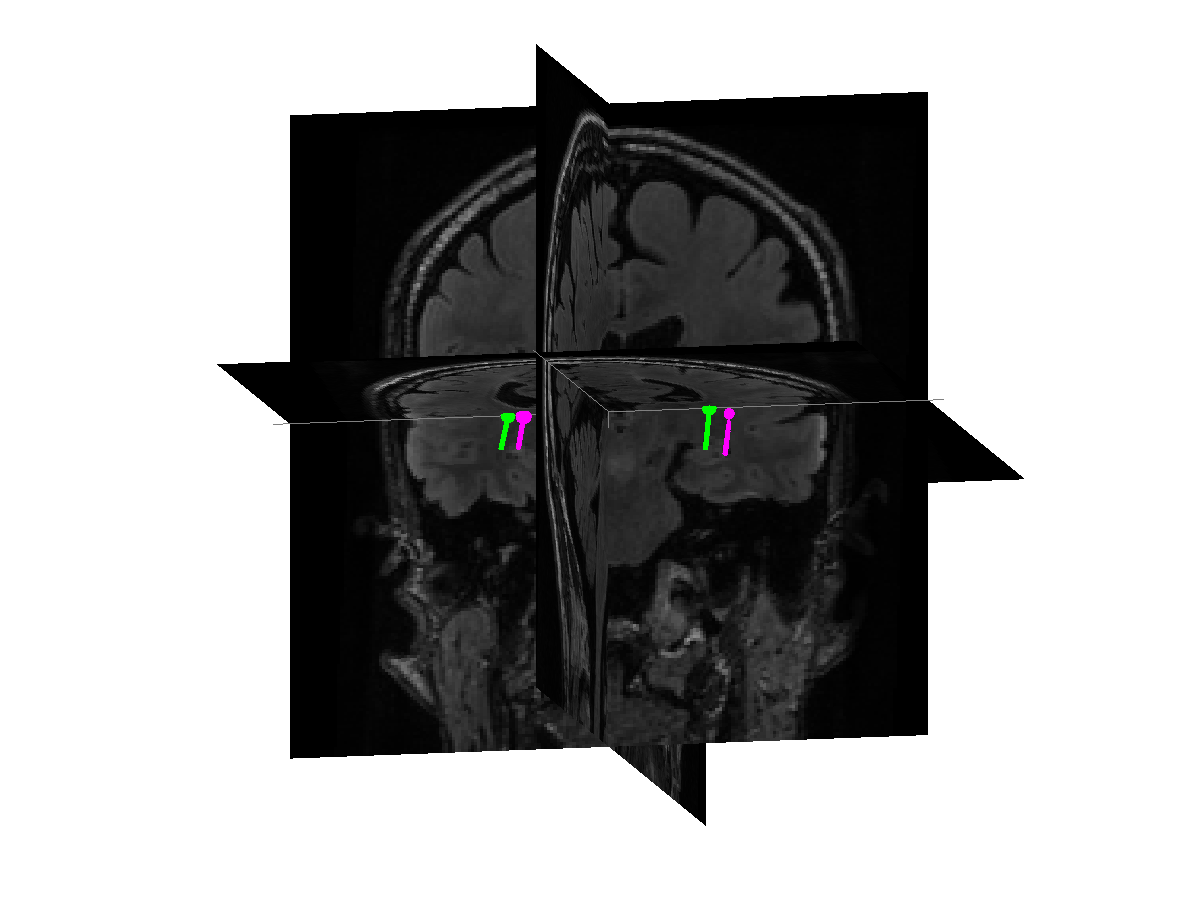You can see that the dipoles have moved a little bit from their original location and that they are not symmetric any more.

Using the dipole locations that we fitted to the rather short time window of the M100, we can estimate the timecourse of activity. That is also done using ft_dipolefitting, now using both cfg.nonlinear=’no’ and cfg.gridsearch=’no’.

``````cfg = [];
cfg.latency = 'all';
cfg.numdipoles = 2;
cfg.symmetry = [];
cfg.nonlinear = 'no';  % use a fixed position
cfg.gridsearch = 'no';
cfg.dip.pos = source_planar.dip.pos;
cfg.channel = {'MEG*2', 'MEG*3'};
cfg.senstype = 'meg';
source_all = ft_dipolefitting(cfg, timelock_all); % estimate the amplitude and orientation
source_std = ft_dipolefitting(cfg, timelock_std); % estimate the amplitude and orientation
source_dev = ft_dipolefitting(cfg, timelock_dev); % estimate the amplitude and orientation
source_dif = ft_dipolefitting(cfg, timelock_dif); % estimate the amplitude and orientation
``````

The orientation and strength of each dipole is represented as a 3*Ntime matrix, with a dipole moment along the x-, y- and z-direction. Since for each timepoint you have a [Qx Qy Qz] vector, which changes over time, you can also consider this as a vector that rotates over time.

``````figure
subplot(3,1,1); title('left: std & dev')
hold on
plot(source_std.time, source_std.dip.mom(1:3,:), '-')
legend({'x', 'y', 'z'});
plot(source_dev.time, source_dev.dip.mom(1:3,:), '.-')
axis([-0.1 0.4 -40e-3 40e-3])
grid on

subplot(3,1,2); title('right: std & dev')
hold on
plot(source_std.time, source_std.dip.mom(4:6,:), '-')
legend({'x', 'y', 'z'});
plot(source_dev.time, source_dev.dip.mom(4:6,:), '.-')
axis([-0.1 0.4 -40e-3 40e-3])
grid on

subplot(3,1,3); title('dif = dev - std')
hold on
plot(source_dif.time, source_dif.dip.mom(1:3,:), '-');
legend({'x', 'y', 'z'});
plot(source_dif.time, source_dif.dip.mom(4:6,:), '-');
axis([-0.1 0.4 -40e-3 40e-3])
grid on

print -dpng natmeg_dip_timeseries.png
``````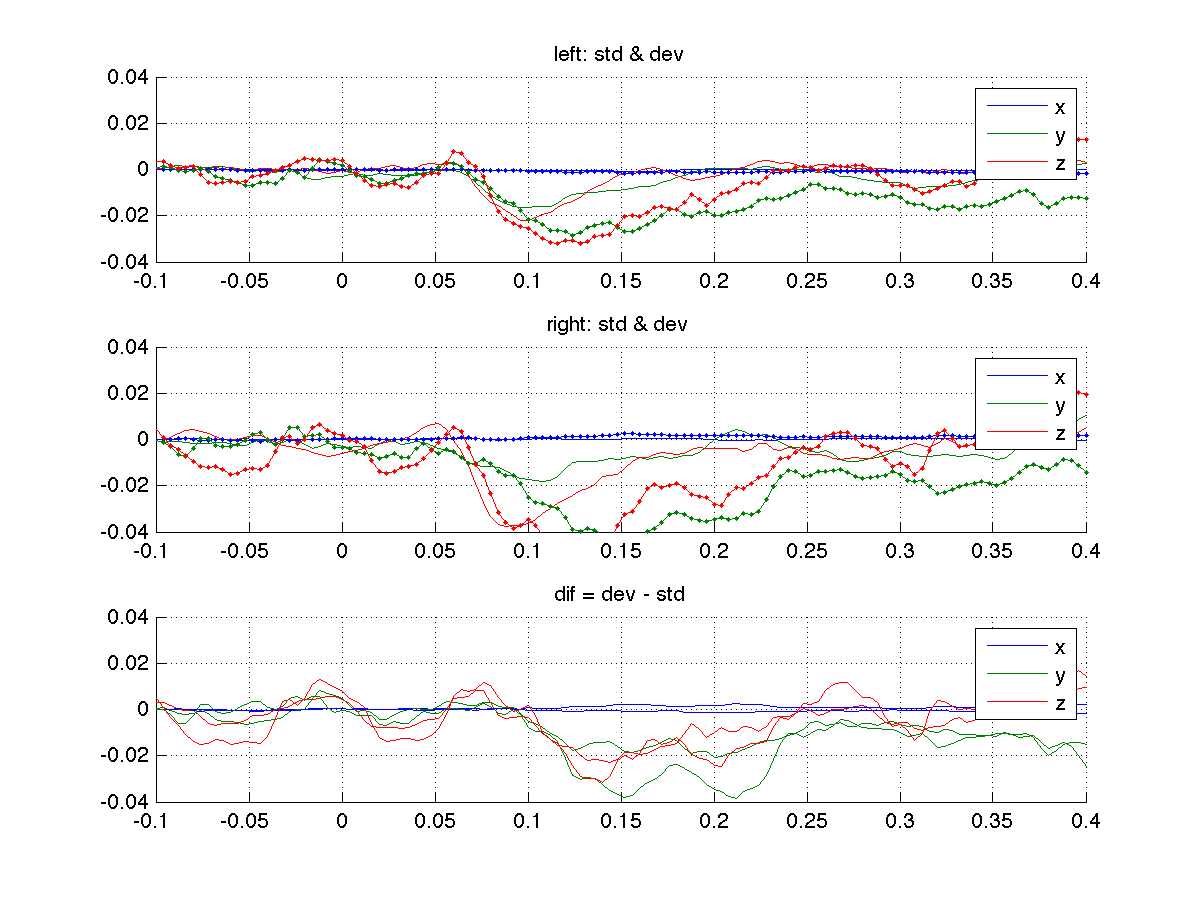Besides comparing the timecourse of the activity between the two conditions, we could also ask whether the activity is at a different location.

``````cfg = [];
cfg.numdipoles = 2;
cfg.symmetry = 'x';
cfg.gridsearch = 'no';
cfg.dip.pos = source_planar.dip.pos;
cfg.channel = {'MEG*2', 'MEG*3'};
cfg.senstype = 'meg';
cfg.latency = [0.080 0.100];
source_all = ft_dipolefitting(cfg, timelock_all);
source_std = ft_dipolefitting(cfg, timelock_std);
source_dev = ft_dipolefitting(cfg, timelock_dev);
``````

The MMN activity starts at about 150 ms, hence we fit that in a slightly later time window.

``````cfg.latency = [0.150 0.180];
source_dif = ft_dipolefitting(cfg, timelock_dif);
``````

We can plot the dipoles together in 3D. Note the color-coding that is used to distinguish the different dipoles.

``````figure
hold on

ft_plot_dipole(source_all.dip.pos(1,:), mean(source_all.dip.mom(1:3,:),2), 'color', 'r')
ft_plot_dipole(source_all.dip.pos(2,:), mean(source_all.dip.mom(4:6,:),2), 'color', 'r')

ft_plot_dipole(source_std.dip.pos(1,:), mean(source_std.dip.mom(1:3,:),2), 'color', 'g')
ft_plot_dipole(source_std.dip.pos(2,:), mean(source_std.dip.mom(4:6,:),2), 'color', 'g')

ft_plot_dipole(source_dev.dip.pos(1,:), mean(source_dev.dip.mom(1:3,:),2), 'color', 'b')
ft_plot_dipole(source_dev.dip.pos(2,:), mean(source_dev.dip.mom(4:6,:),2), 'color', 'b')

ft_plot_dipole(source_dif.dip.pos(1,:), mean(source_dif.dip.mom(1:3,:),2), 'color', 'y')
ft_plot_dipole(source_dif.dip.pos(2,:), mean(source_dif.dip.mom(4:6,:),2), 'color', 'y')

pos = mean(source_std.dip.pos,1);
ft_plot_slice(mri_resliced_cm.anatomy, 'transform', mri_resliced_cm.transform, 'location', pos, 'orientation', [1 0 0], 'resolution', 0.1)
ft_plot_slice(mri_resliced_cm.anatomy, 'transform', mri_resliced_cm.transform, 'location', pos, 'orientation', [0 1 0], 'resolution', 0.1)
ft_plot_slice(mri_resliced_cm.anatomy, 'transform', mri_resliced_cm.transform, 'location', pos, 'orientation', [0 0 1], 'resolution', 0.1)

ft_plot_crosshair(pos, 'color', [1 1 1]/2);

axis tight
axis off

print -dpng natmeg_dip_sourcedif.png
``````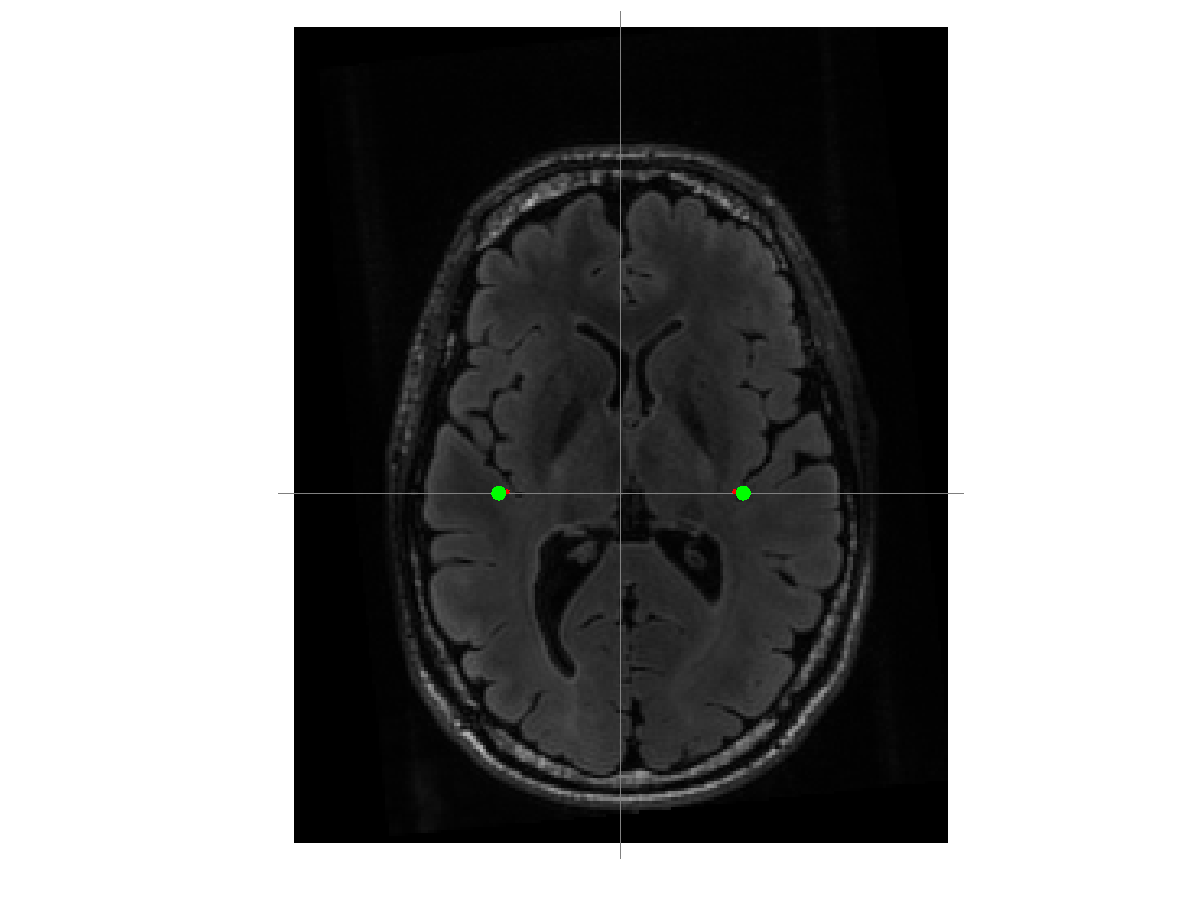The dipole positions are not exactly the same. Explain the difference in the dipole position and how the MMN might contribute to the dipole position of the deviant being shifted inward.

Rather than assuming that the dipole position is fixed over a certain time-window, we can also fit a dipole to each topography separately, i.e. to each sample in the data. Since this results in a dipole position that is different over time, this is also referred to as a “moving dipole” model.

``````cfg = [];
cfg.model = 'moving'; % default is rotating
cfg.latency = [0.070 0.140];
cfg.numdipoles = 2;
cfg.gridsearch = 'no';
cfg.dip.pos = source_planar.dip.pos;
cfg.channel = {'MEG*2', 'MEG*3'};
cfg.senstype = 'meg';
source = ft_dipolefitting(cfg, timelock_std);

% copy the time-varying position of the two dipoles into a single matrix for convenience.
for i=1:numel(source.dip)
pos1(i,:) = source.dip(i).pos(1,:);
pos2(i,:) = source.dip(i).pos(2,:);
end

figure
hold on

plot3(pos1(:,1), pos1(:,2), pos1(:,3), 'r.')
plot3(pos2(:,1), pos2(:,2), pos2(:,3), 'g.')

pos = (mean(pos1, 1) + mean(pos2, 1))/2;

ft_plot_slice(mri_resliced_cm.anatomy, 'transform', mri_resliced_cm.transform, 'location', pos, 'orientation', [1 0 0], 'resolution', 0.1)
ft_plot_slice(mri_resliced_cm.anatomy, 'transform', mri_resliced_cm.transform, 'location', pos, 'orientation', [0 1 0], 'resolution', 0.1)
ft_plot_slice(mri_resliced_cm.anatomy, 'transform', mri_resliced_cm.transform, 'location', pos, 'orientation', [0 0 1], 'resolution', 0.1)

ft_plot_crosshair(pos, 'color', [1 1 1]/2);

axis tight
axis off

print -dpng natmeg_dip_moving.png
``````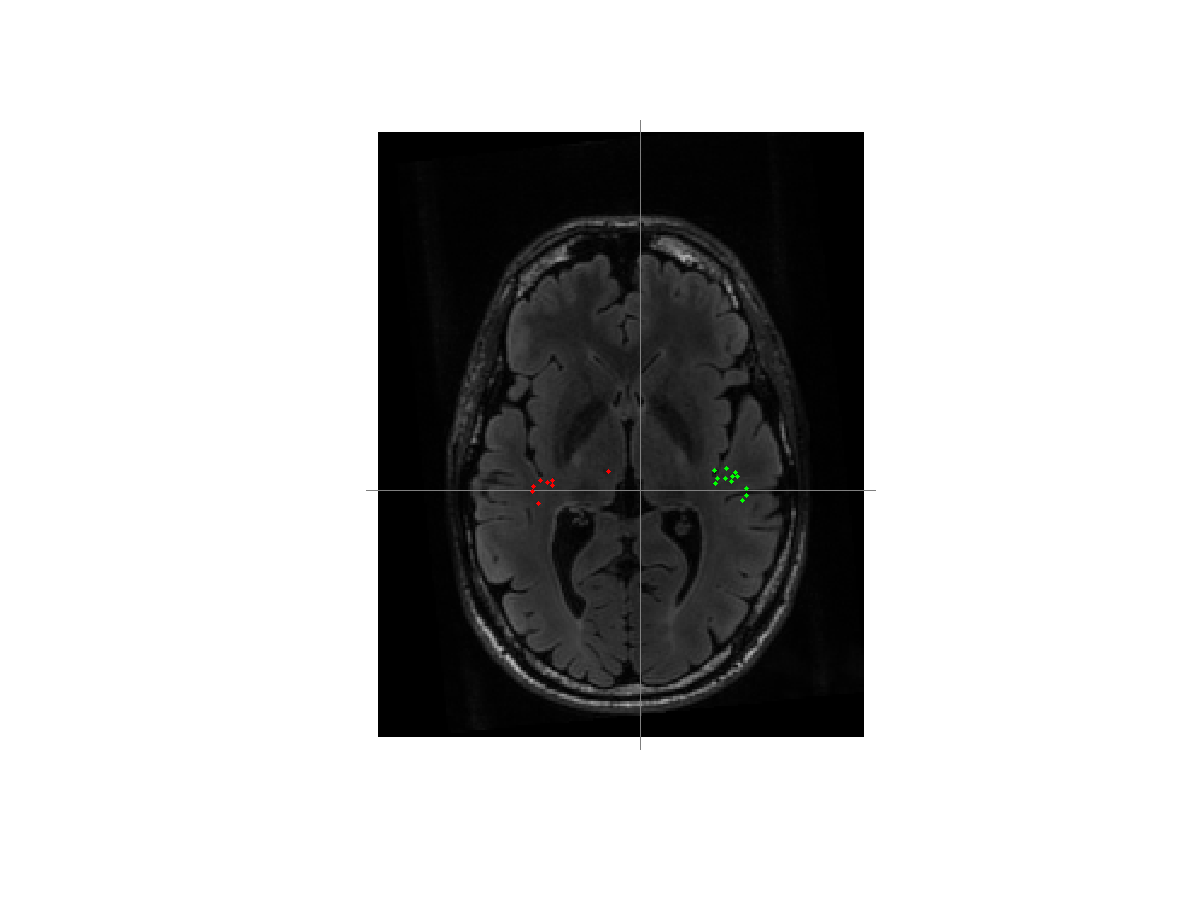### Construct the EEG volume conduction model

The EEG needs a different volume conduction model than the EEG. Previously we already constructed the meshes for the three important compartments of the head.

``````figure
ft_plot_mesh(mesh_brain, 'edgecolor', 'none', 'facecolor', 'r')
ft_plot_mesh(mesh_skull, 'edgecolor', 'none', 'facecolor', 'g')
ft_plot_mesh(mesh_scalp, 'edgecolor', 'none', 'facecolor', 'b')
alpha 0.3
view(132, 14)

print -dpng natmeg_dip_meshorig.png
``````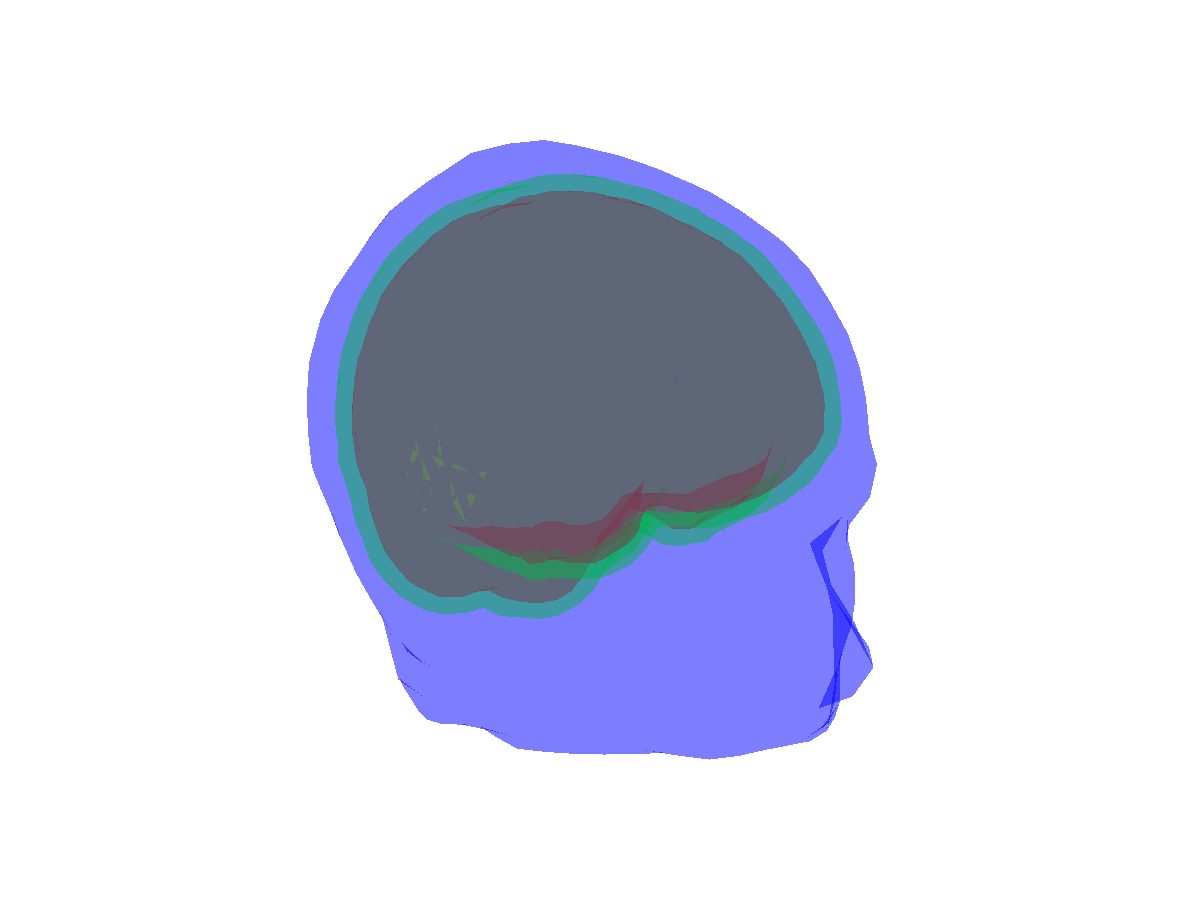If you look carefully, you can identify a problem with the mesh. The BEM requires that the meshes are closed and non-intersecting. The figure shows that over right temporal regions there are some vertices of the skull surface that stick out of the skull. This is due to an overestimation of the skull thickness over the temporal region.

One solution would be to inflate the scalp mesh a bit, i.e. to scale it a bit outward.

``````mesh_scalp_infl = mesh_scalp;
mesh_scalp_infl.pos = 1.10 * mesh_scalp_infl.pos;

figure
ft_plot_mesh(mesh_brain, 'edgecolor', 'none', 'facecolor', 'r')
ft_plot_mesh(mesh_skull, 'edgecolor', 'none', 'facecolor', 'g')
ft_plot_mesh(mesh_scalp_infl, 'edgecolor', 'none', 'facecolor', 'b')
alpha 0.3
view(132, 14)

print -dpng natmeg_dip_meshinfl.png
``````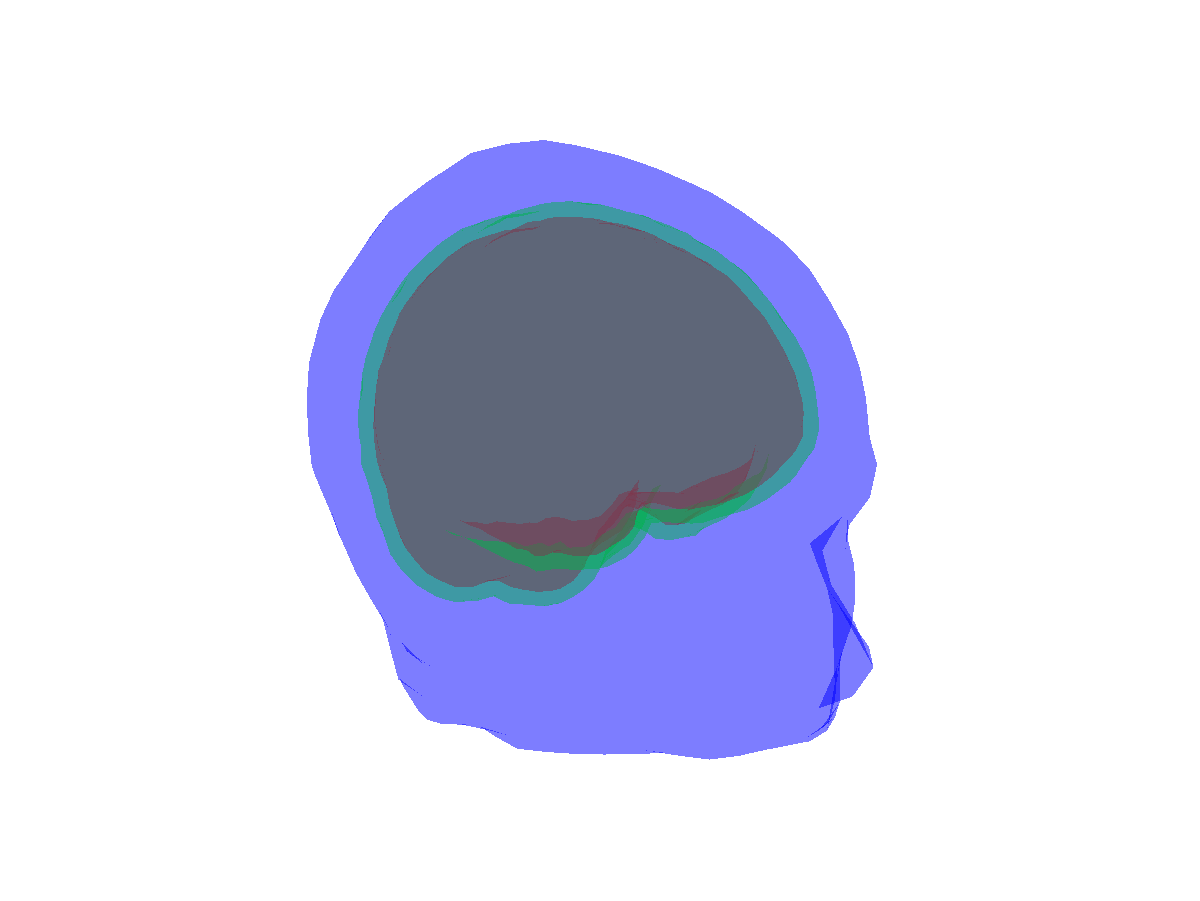This does address the problem, however also causes the skin to become thicker all-over.

A better approach is to return to the segmented anatomical MRI and to use image processing tools to fix the segmentation. The tools we will use are imerode and imdilate. Their effect is demonstrated in one of the frequently asked questions.

Here we will divert from FieldTrip and use some off-the-shelf MATLAB code. We start by copying the 3-D arrays of the segmentation into three separate variables. Using |, i.e. the logical “OR” operator, we can combine the brain skull and scalp into filled volumes.

``````binary_brain = mri_segmented.brain;
binary_skull = mri_segmented.skull | binary_brain;
binary_scalp = mri_segmented.scalp | binary_brain | binary_skull;
``````

The following code demonstrates the effect of the imdilate function. It makes four figures, starting from the original segmentation.

``````close all

% using ft_sourceplot I identified the cross-section with voxel
% indices [107 100 100] where the problem is visible and I will
% plot that intersection multiple times

figure(1)
tmp = binary_scalp + binary_skull + binary_brain;
imagesc(squeeze(tmp(:,:,100)));
print -dpng natmeg_dip_segorg.png

% use IMDILATE to inflate the segmentation
binary_scalp = imdilate(binary_scalp, strel_bol(1));

figure(2)
tmp = binary_scalp + binary_skull + binary_brain;
imagesc(squeeze(tmp(:,:,100)));
print -dpng natmeg_dip_segdil1.png

% use IMDILATE to inflate the segmentation a bit more
binary_scalp = imdilate(binary_scalp, strel_bol(1));

figure(3)
tmp = binary_scalp + binary_skull + binary_brain;
imagesc(squeeze(tmp(:,:,100)));
print -dpng natmeg_dip_segdil2.png

% revert to the oriiginal binary_scalp
binary_scalp = mri_segmented.scalp + binary_skull;

% use boolean logic together with IMERODE
binary_skull = binary_skull & imerode(binary_scalp, strel_bol(2)); % fully contained inside eroded scalp
binary_brain = binary_brain & imerode(binary_skull, strel_bol(2)); % fully contained inside eroded skull

figure(4)
tmp = binary_scalp + binary_skull + binary_brain;
imagesc(squeeze(tmp(:,:,100)));
print -dpng natmeg_dip_segbool.png
``````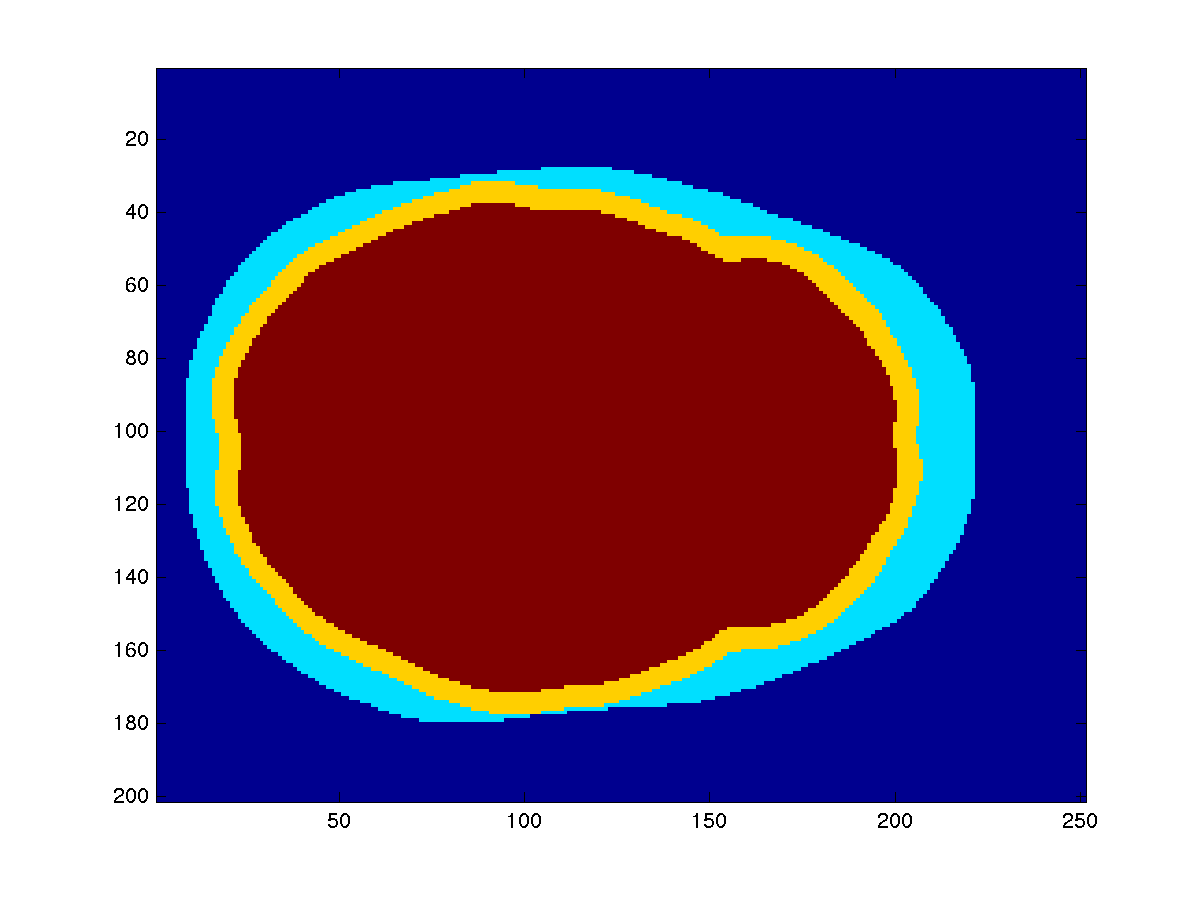_The original segmentation _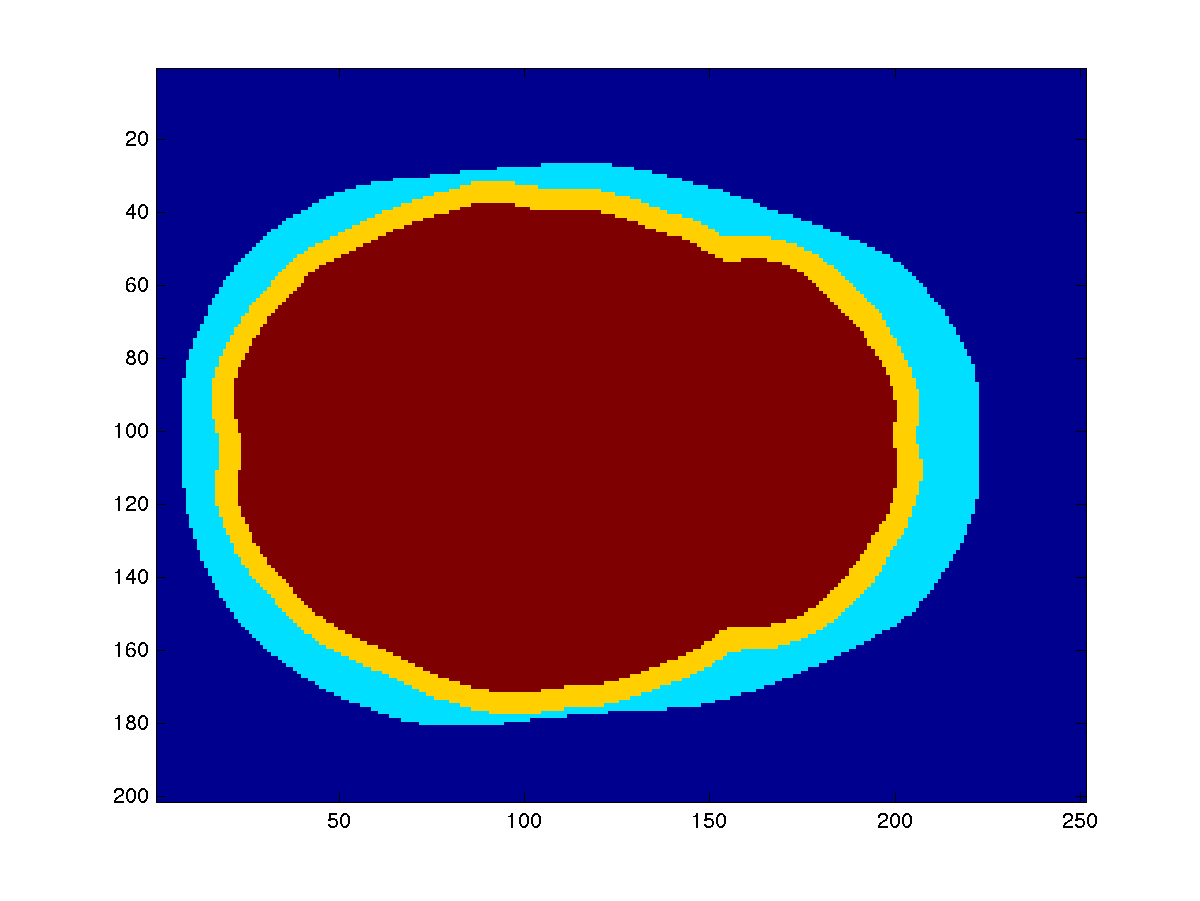_After dilation of 1 voxel _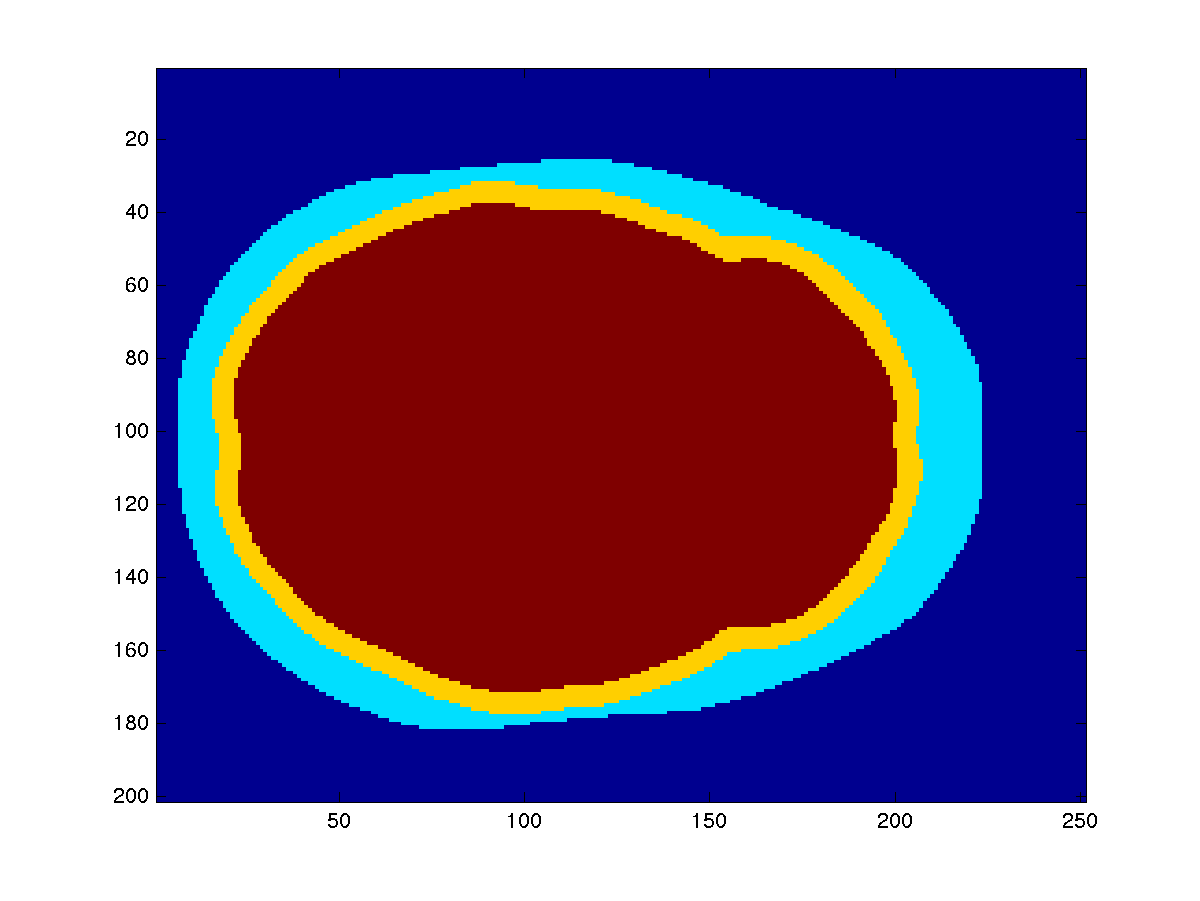_After dilation of 2 voxels _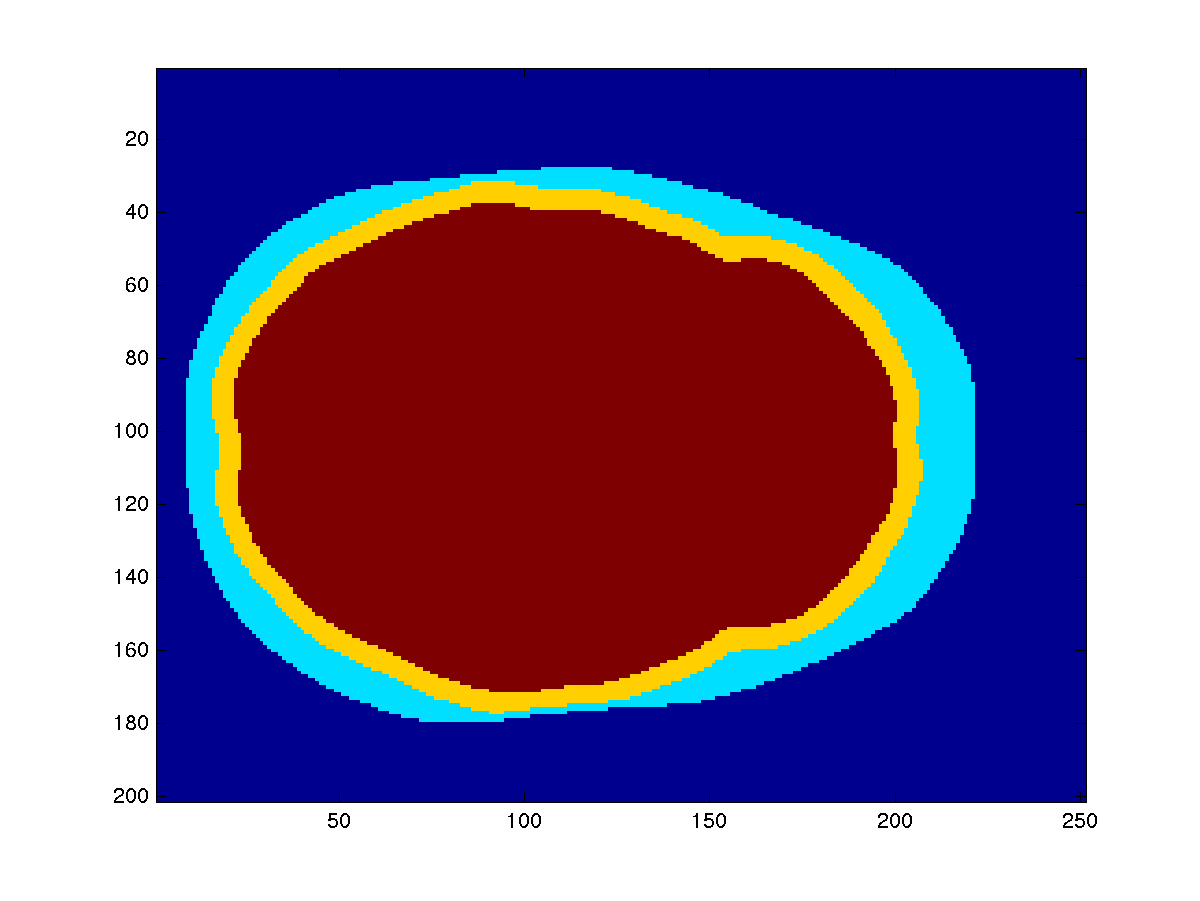_The final segmentation _

Using a combination of imerode and Boolean locic with the “AND” operator, we can make a segmentation of the scalp, skull and skin that is not inflated.

Compare the four figures and toggle back and forth. Can you see the effect of the dilation on the outside of the scalp?

Having completed the manual refinement of the segmentation on the three temporary arrays, we copy them back into the original segmentation structure.

``````mri_segmented2 = mri_segmented;
% insert the updated binary volumes, taking out the center part for skull and scalp
mri_segmented2.brain    = binary_brain;
mri_segmented2.skull    = binary_skull & ~binary_brain;
mri_segmented2.scalp    = binary_scalp & ~binary_brain & ~binary_skull;
mri_segmented2.combined = binary_scalp + binary_skull + binary_brain; % only for plotting

save mri_segmented2 mri_segmented2
``````

The “combined” field contains the sum of the three segmentations, which means that it is 1 for scalp, 2 for skull and 3 for brain. This allows us to look at all three segmentations at once in ft_sourceplot.

``````cfg = [];
cfg.funparameter = 'combined';
cfg.funcolormap = 'jet';
ft_sourceplot(cfg, mri_segmented2);
``````

The trick with the “combined” field is a bit of a hack, and we should remove it again from the segmentation structure.

``````% this has to be removed, otherwise ft_prepare_mesh gets confused
mri_segmented2 = rmfield(mri_segmented2, 'combined');
``````

Using the updated segmentation, we reconstruct the three triangulated meshes.

``````cfg = [];
cfg.method = 'projectmesh';
cfg.tissue = 'brain';
cfg.numvertices = 3000;
mesh_eeg(1) = ft_prepare_mesh(cfg, mri_segmented2);

cfg.tissue = 'skull';
cfg.numvertices = 2000;
mesh_eeg(2) = ft_prepare_mesh(cfg, mri_segmented2);

cfg.tissue = 'scalp';
cfg.numvertices = 1000;
mesh_eeg(3) = ft_prepare_mesh(cfg, mri_segmented2);

figure
ft_plot_mesh(mesh_eeg(1), 'edgecolor', 'none', 'facecolor', 'r')
ft_plot_mesh(mesh_eeg(2), 'edgecolor', 'none', 'facecolor', 'g')
ft_plot_mesh(mesh_eeg(3), 'edgecolor', 'none', 'facecolor', 'b')
alpha 0.3

save mesh_eeg mesh_eeg
``````

The three meshes are combined in one struct-array and used as input to ft_prepare_headmodel. We also have to specify the conductivity of each of the tissue types.

``````cfg = [];
cfg.method = 'bemcp';
cfg.conductivity = [1 1/20 1].*0.33; % brain, skull, scalp

``````

Here we’ve set the ratio of conductivity between the different tissue types to [1 1/20 1]. What would happen if we would change the ratio to: [1 1/80 1]? See What is the conductivity of the brain, CSF, skull and skin tissue?

### Process the EEG data

We are going to process the EEG data in much the same way as the MEG data. As you are already familiar with how to do this you can speed through this section. Again, this requires the custom trial function trialfun_oddball_stimlocked.m to be on your MATLAB path.

#### Segment and read the EEG data

First we are going to read the data into trial

``````cfg = [];
cfg.dataset = dataset;
cfg.trialdef.prestim        = 0.2;
cfg.trialdef.poststim       = 0.4;
cfg.trialdef.rsp_triggers   = [256 4096];
cfg.trialdef.stim_triggers  = [1 2];
cfg.trialfun                = 'trialfun_oddball_stimlocked';

cfg = ft_definetrial(cfg);

cfg.continuous    = 'yes';
cfg.hpfilter      = 'no';
cfg.detrend       = 'no';
cfg.demean        = 'yes';
cfg.baselinewindow = [-inf 0];
cfg.dftfilter     = 'yes';
cfg.dftfreq       = [50 100];
cfg.lpfilter      = 'yes';
cfg.lpfreq        = 120;
cfg.channel       = 'EEG';
cfg.precision     = 'single';

data_eeg = ft_preprocessing(cfg);

save data_eeg data_eeg
``````

As before we are going to check for, and remove bad trial

``````cfg = [];
cfg.method = 'summary';
cfg.keepchannel = 'no';
cfg.preproc.reref = 'yes';
cfg.preproc.refchannel = 'all';
data_eeg_clean = ft_rejectvisual(cfg, data_eeg);
``````

The EEG forward model is computed with an common average reference. Consequently, the EEG data that you want to fit also should be average referenced. Whenever you remove a (bad) channel from the data, you have to recompute the common average reference in the EEG data.

``````cfg = [];
cfg.reref = 'yes';
cfg.refchannel = 'all';
data_eeg_reref = ft_preprocessing(cfg, data_eeg_clean);

save data_eeg_reref data_eeg_reref
``````

#### Compute the time-locked average

We will now calculate the ERPs on which we are going to fit the dipole

``````cfg = [];
timelock_eeg_all = ft_timelockanalysis(cfg, data_eeg_reref);

cfg.trials = find(data_eeg_reref.trialinfo==1);
timelock_eeg_std = ft_timelockanalysis(cfg, data_eeg_reref);

cfg.trials = find(data_eeg_reref.trialinfo==2);
timelock_eeg_dev = ft_timelockanalysis(cfg, data_eeg_reref);
``````

Before continuing lets just have a quick look whether we processed our data correctly, the following code should produce a familiar imag

``````cfg = [];
cfg.layout = 'natmeg_customized_eeg1005.lay';
ft_multiplotER(cfg, timelock_eeg_std, timelock_eeg_dev);

print -dpng natmeg_dip_meg_multiplot.png

cfg = [];
cfg.parameter = 'avg';
cfg.operation = 'x1 - x2';
timelock_eeg_dif = ft_math(cfg, timelock_eeg_dev, timelock_eeg_std);

cfg = [];
cfg.layout = 'natmeg_customized_eeg1005.lay';
ft_multiplotER(cfg, timelock_eeg_dif);
``````

### Compare the EEG and MEG dipole fits

Now we are actually able to do the dipole fitting on the EEG dat

``````cfg = [];
cfg.latency = [0.080 0.110];
cfg.numdipoles = 2;
cfg.symmetry = 'x';
cfg.resolution = 1;
cfg.unit = 'cm';
cfg.gridsearch = 'yes';
cfg.senstype = 'eeg';
cfg.channel = 'all';
source_eeg = ft_dipolefitting(cfg, timelock_eeg_all);
``````

Lets plot the dipoles and see how it compares to our fit of the MEG dat

``````cfg = [];
cfg.location = source_eeg.dip.pos(1,:);
ft_sourceplot(cfg, mri_resliced_cm);

figure

ft_plot_dipole(source_eeg.dip.pos(1,:), mean(source_eeg.dip.mom(1:3,:),2), 'color', 'b')
ft_plot_dipole(source_eeg.dip.pos(2,:), mean(source_eeg.dip.mom(4:6,:),2), 'color', 'b')

ft_plot_dipole(source_mag.dip.pos(1,:), mean(source_mag.dip.mom(1:3,:),2), 'color', 'r')
ft_plot_dipole(source_mag.dip.pos(2,:), mean(source_mag.dip.mom(4:6,:),2), 'color', 'r')

ft_plot_dipole(source_planar.dip.pos(1,:), mean(source_planar.dip.mom(1:3,:),2), 'color', 'g')
ft_plot_dipole(source_planar.dip.pos(2,:), mean(source_planar.dip.mom(4:6,:),2), 'color', 'g')

pos = mean(source_eeg.dip.pos,1);
% pos = source_eeg.dip.pos(1,:); % use another crossection for the MRI

ft_plot_slice(mri_resliced_cm.anatomy, 'transform', mri_resliced_cm.transform, 'location', pos, 'orientation', [1 0 0], 'resolution', 0.1)
ft_plot_slice(mri_resliced_cm.anatomy, 'transform', mri_resliced_cm.transform, 'location', pos, 'orientation', [0 1 0], 'resolution', 0.1)
ft_plot_slice(mri_resliced_cm.anatomy, 'transform', mri_resliced_cm.transform, 'location', pos, 'orientation', [0 0 1], 'resolution', 0.1)

ft_plot_crosshair(pos, 'color', [1 1 1]/2);

axis tight
axis off
``````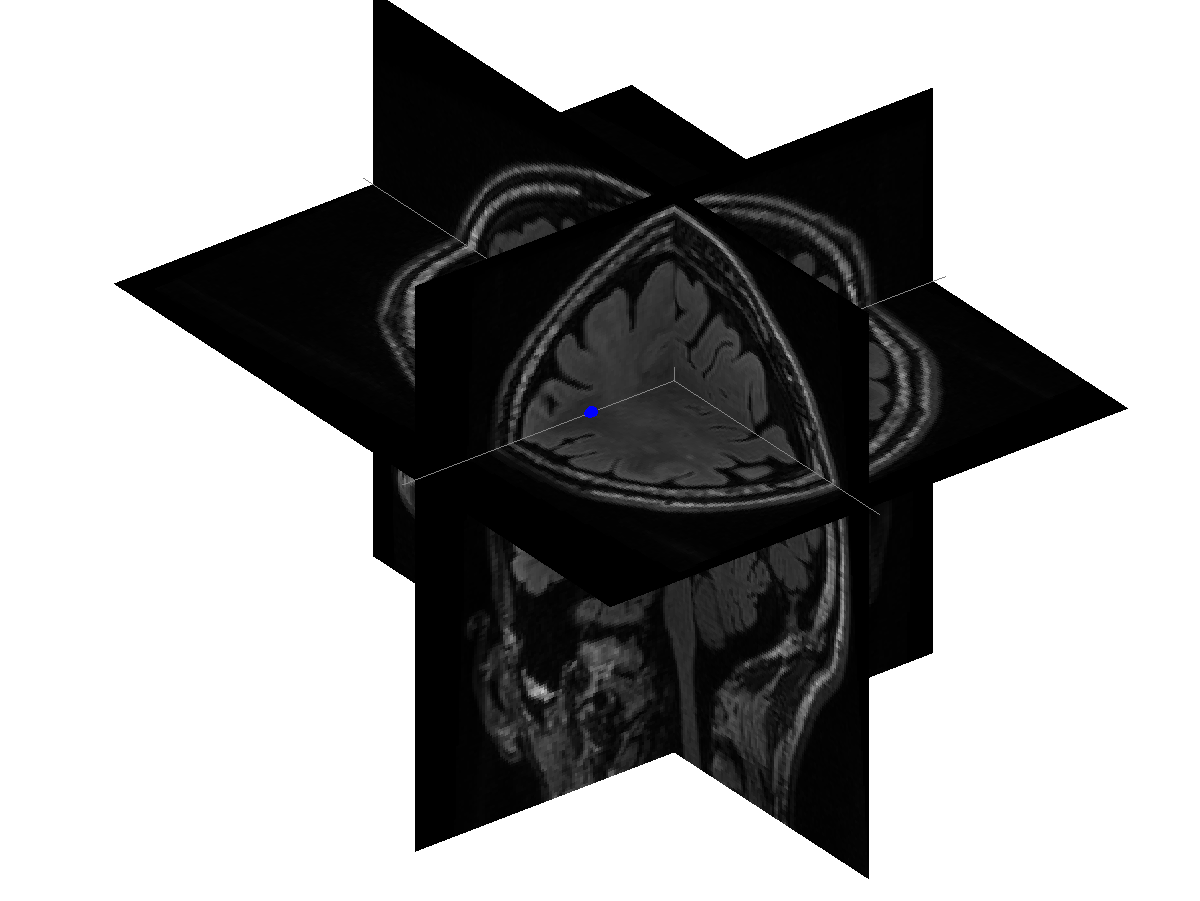The EEG dipole fit is not so trustworthy as the MEG dipole fit. We can try to release the symmetry constraint and fit the 2-dipole mode, starting from the symmetric position as initial guess.

``````cfg = [];
cfg.latency = [0.080 0.110];
cfg.numdipoles = 2;
cfg.dip.pos = source_eeg.dip.pos;
cfg.gridsearch = 'no';
cfg.nonlinear = 'yes';
cfg.senstype = 'eeg';
cfg.channel = 'all';
source_eeg2 = ft_dipolefitting(cfg, timelock_eeg_all);

figure

ft_plot_dipole(source_eeg.dip.pos(1,:), mean(source_eeg.dip.mom(1:3,:),2), 'color', 'b')
ft_plot_dipole(source_eeg.dip.pos(2,:), mean(source_eeg.dip.mom(4:6,:),2), 'color', 'b')

ft_plot_dipole(source_eeg2.dip.pos(1,:), mean(source_eeg2.dip.mom(1:3,:),2), 'color', 'm')
ft_plot_dipole(source_eeg2.dip.pos(2,:), mean(source_eeg2.dip.mom(4:6,:),2), 'color', 'm')

pos = mean(source_eeg.dip.pos,1);
% pos = source_eeg.dip.pos(1,:); % alternative crossection for the MRI

ft_plot_slice(mri_resliced_cm.anatomy, 'transform', mri_resliced_cm.transform, 'location', pos, 'orientation', [1 0 0], 'resolution', 0.1)
ft_plot_slice(mri_resliced_cm.anatomy, 'transform', mri_resliced_cm.transform, 'location', pos, 'orientation', [0 1 0], 'resolution', 0.1)
ft_plot_slice(mri_resliced_cm.anatomy, 'transform', mri_resliced_cm.transform, 'location', pos, 'orientation', [0 0 1], 'resolution', 0.1)

ft_plot_crosshair(pos, 'color', [1 1 1]/2);

axis tight
axis off
``````

How does this fit compare to the previous? Can you explain the difference?

## Summary and conclusion

We demonstrated how to use dipole fitting to estimate the location and timecourse of the auditory evoked fields and the mismatch negativity. We computed the optimal dipole fits using different constraints (i.e. assumptions) on the dipole models. The fitted dipole position of the AEF in the “deviant” condition differs from the position in the “standard” condition, which can be explained by an additional set of sources in the deviant condition at a slightly deeper location.

This tutorial demonstrates how you can use different assumptions to get stable and meaningful dipole fit locations. However, it also demonstrates that in the dipole fitting procedure there are many choices than can be made, and that it is not easy to get all parameters right for a meaningful dipole fit solution. This explains why commercial software packages such as BESA have elaborate graphical user interfaces in which you can more easily explore the effect of the constraints on the dipoles, and why sequential dipole fitting strategies are required to construct dipole models for more complicated source configurations.

More details on constructing volume conduction models of the head can be found here for MEG and here for EEG. Other tutorials are available that demonstrate the MNE and Beamformer methods. An alternative method for computing the activity time series at regions of interest using beamformers is described here.